81% OFF online library of tutorials for mastering Google Sheets

One time purchase \$199

Hey there! Register a new account to access the full suite of features, tutorials, and more..

# VLOOKUP

Use VLOOKUP() when you want a specific value in a column of data. Use VLOOKUP() when you want to retrieve information from a different column based on that value. Great for creating amazing summaries, dynamic reports, or managing inventory

# SUMIF

When you need to calculate the sum of values in a range of cells that meet specific criteria, use SUMIF(). Great to use when you need to filter data. For instance, you can use it to sum all the revenue generated from a particular region, all the orders placed by a specific customer, or all the products with a certain attribute.

# COUNTIF

Use COUNTIF() when you're trying to figure out frequency. Learn how to use COUNTIF in Google Sheets. Also check out it's possible alternative: SUMIF. Depending on what you're trying to do, COUNTIF could be your solution or not.

# IF

Powerful and mysterious. Use IF() to make your spreadsheets gateways. Learn how to use IF in Google Sheets. Also check out it's oft-partner ISBLANK()

# FILTER

the FILTER() formula in Google Sheets allows you to filter data based on a set of criteria. Learn how to use FILTER in Google Sheets. Also check out it's possible combination alternative: INDEX/SORT.

# INDEX

A seemingly innocent and useless formula can be so powerful. INDEX pairs nicely with MATCH to replace VLOOKUP. And it's uses are almost endless. Learn more about the INDEX() formula in Google Sheets.

# VLOOKUP

Powerful vlookup and easy to use. Learn how to use VLOOKUP in Google Sheets. Also check out it's possible alternative: INDEX/MATCH.

# SUMIF

Don't know the syntax, or how to use SUMIF? Learn how to use SUMIF in Google Sheets. Also check out it's possible alternative: COUNTIF. Depending on what you're trying to do, SUMIF could be your solution or not.

# CONCATENATE

Probably the most easily misspelled formula in all of Google Sheets. Learn how to use CONCATENATE in Google Sheets. Also check out it's possible alternative: JOIN.

# 515Formulas

Every Formula in Google Sheets, From ABS to ZTEST, including Vlookup and, my favorite, IF()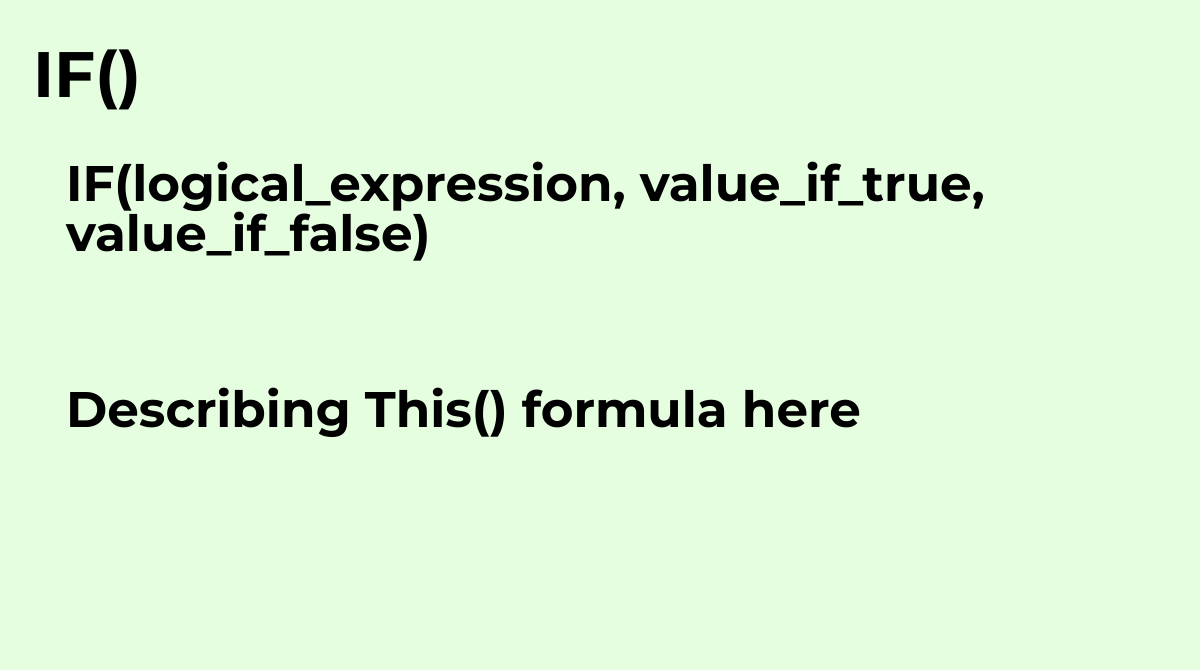Returns one value if a logical expression is `TRUE` and another if it is `FALSE`.What are the com...
`IF(logical_expression, value_if_true, value_if_false)`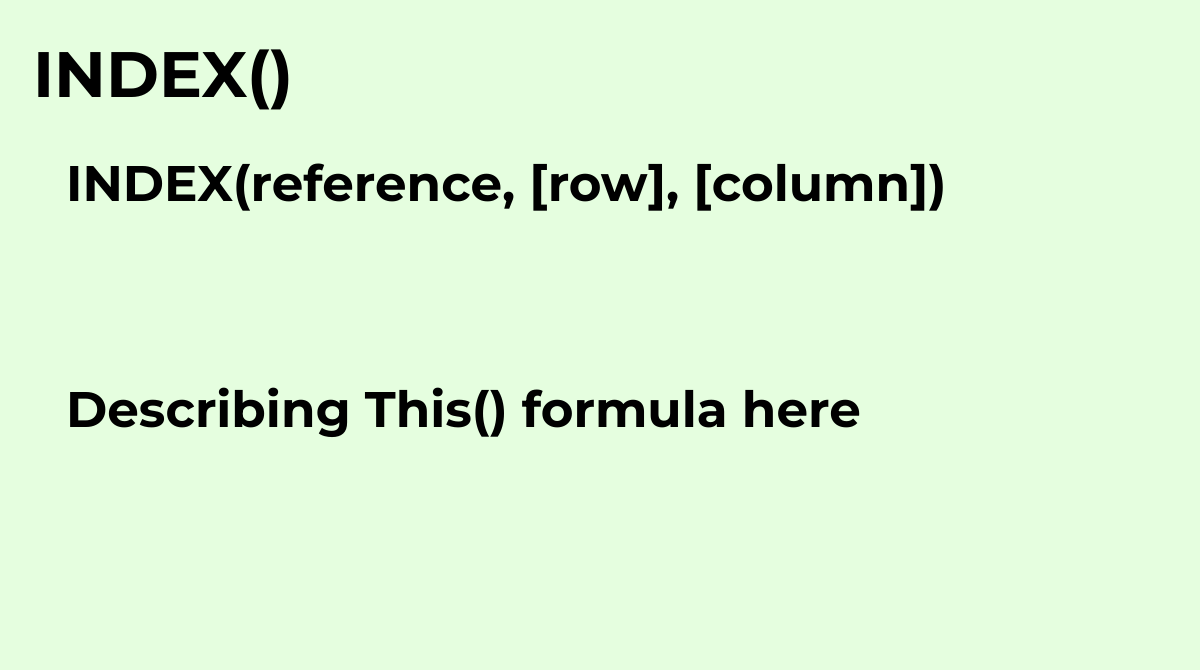Returns the content of a cell, specified by row and column offset.Common questions about the INDE...
`INDEX(reference, [row], [column])`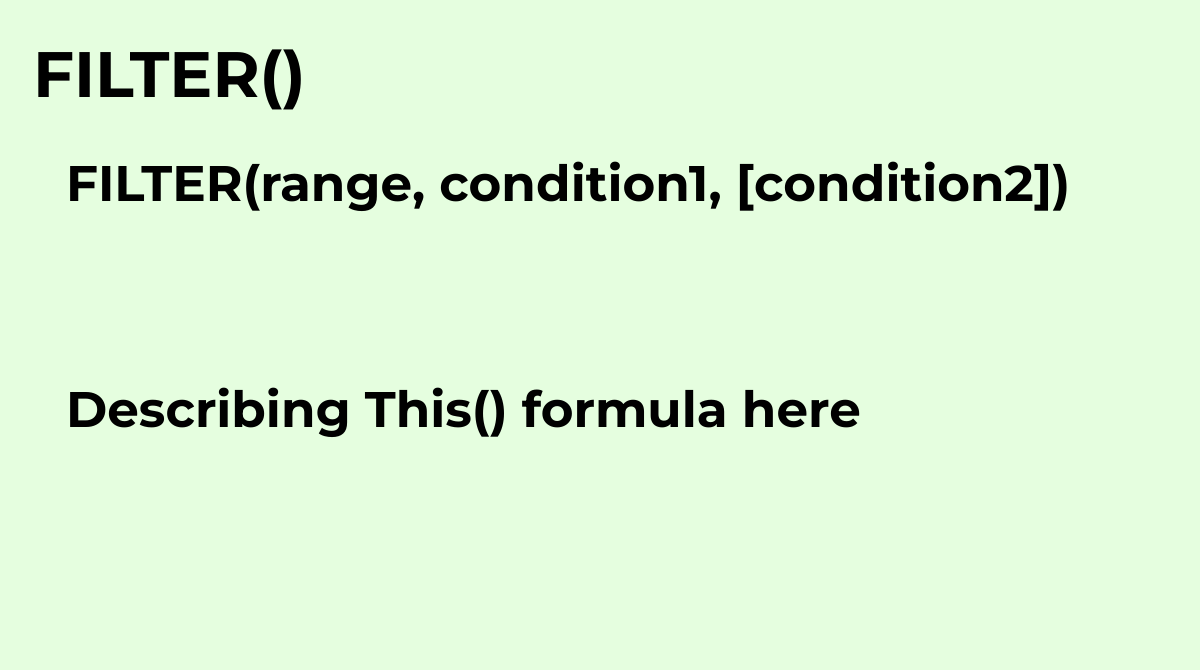Returns a filtered version of the source range, returning only rows or columns which meet the spe...
`FILTER(range, condition1, [condition2])`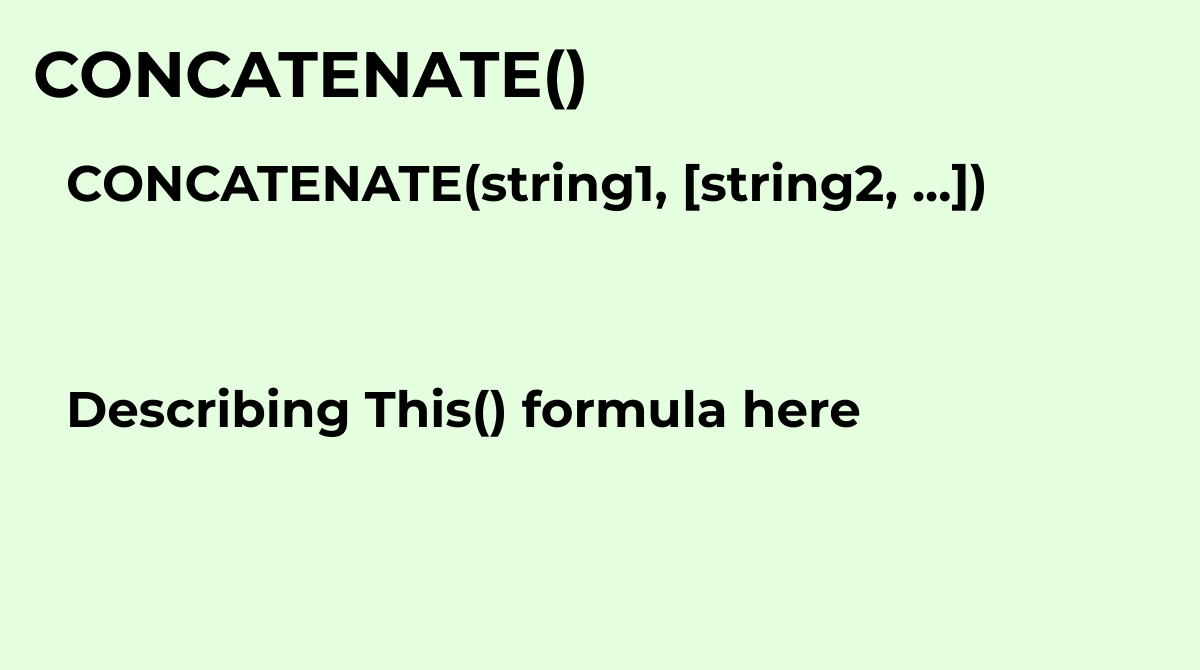Appends strings to one another.Common questions about the CONCATENATE formula:What is the CONCATE...
`CONCATENATE(string1, [string2, ...])`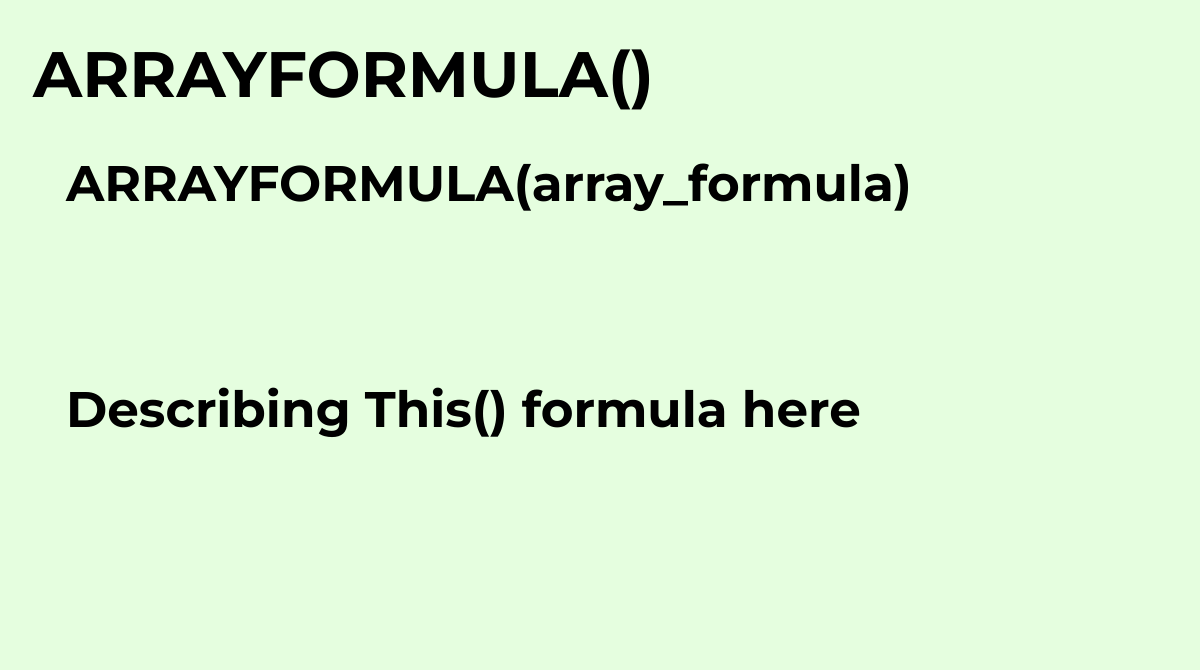Enables the display of values returned from an array formula into multiple rows and/or columns an...
`ARRAYFORMULA(array_formula)`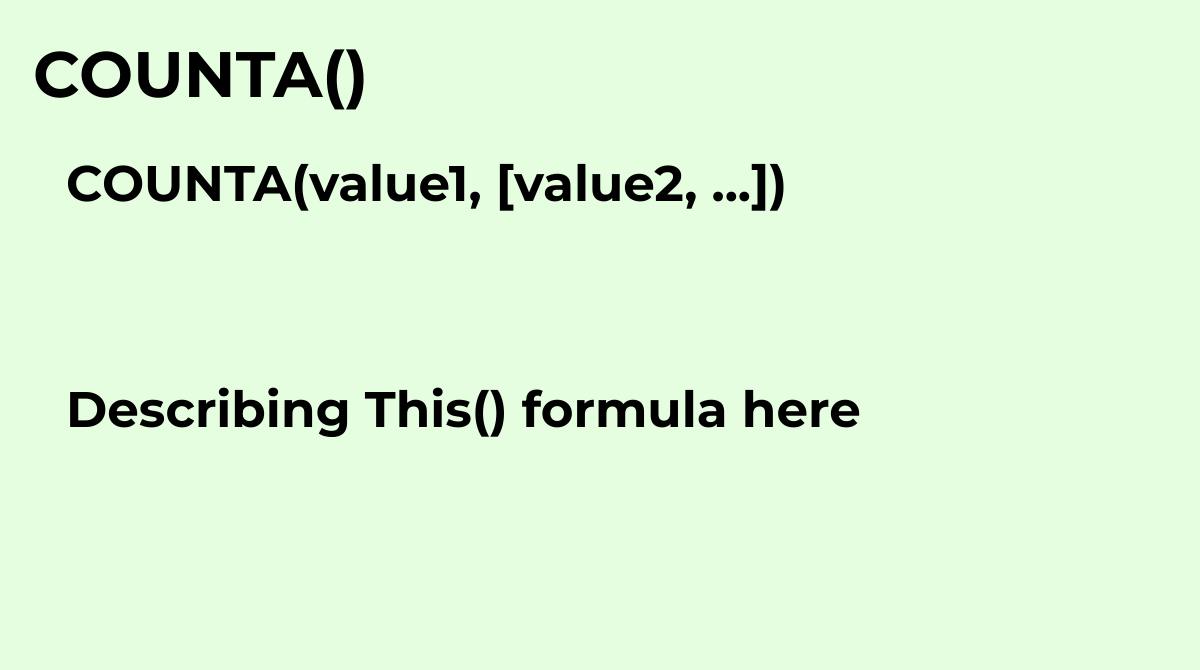Returns a count of the number of values in a dataset.What are the common questions about the COUN...
`COUNTA(value1, [value2, ...])`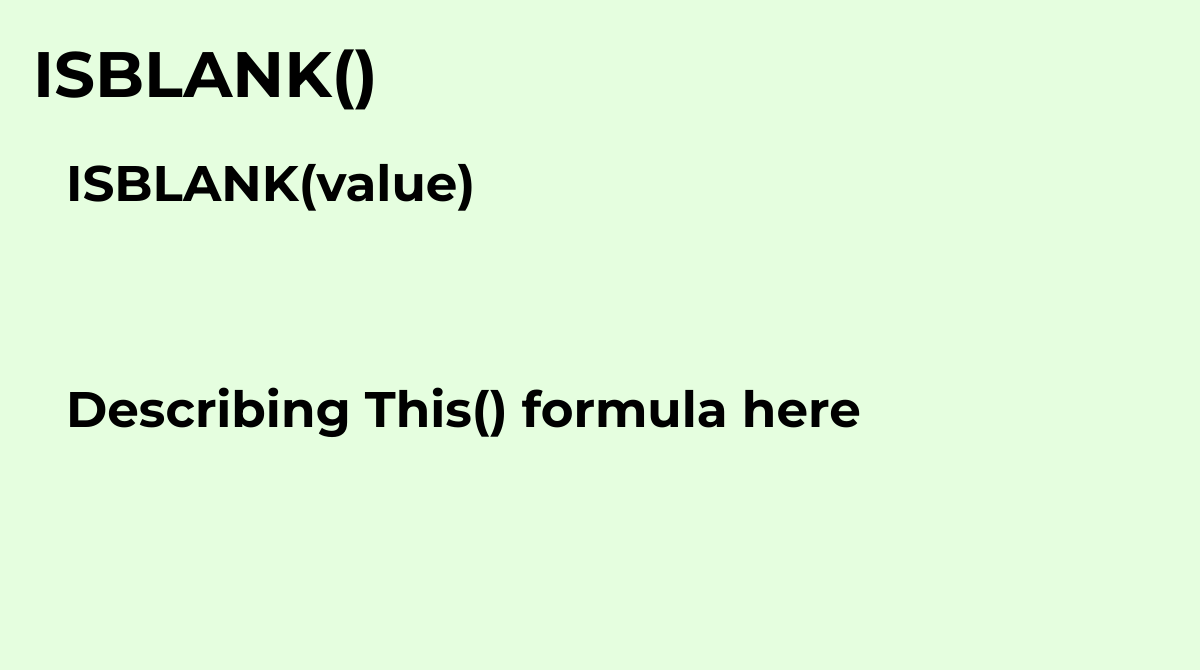Checks whether the referenced cell is empty.Common Questions about the ISBLANK Formula: What is t...
`ISBLANK(value)`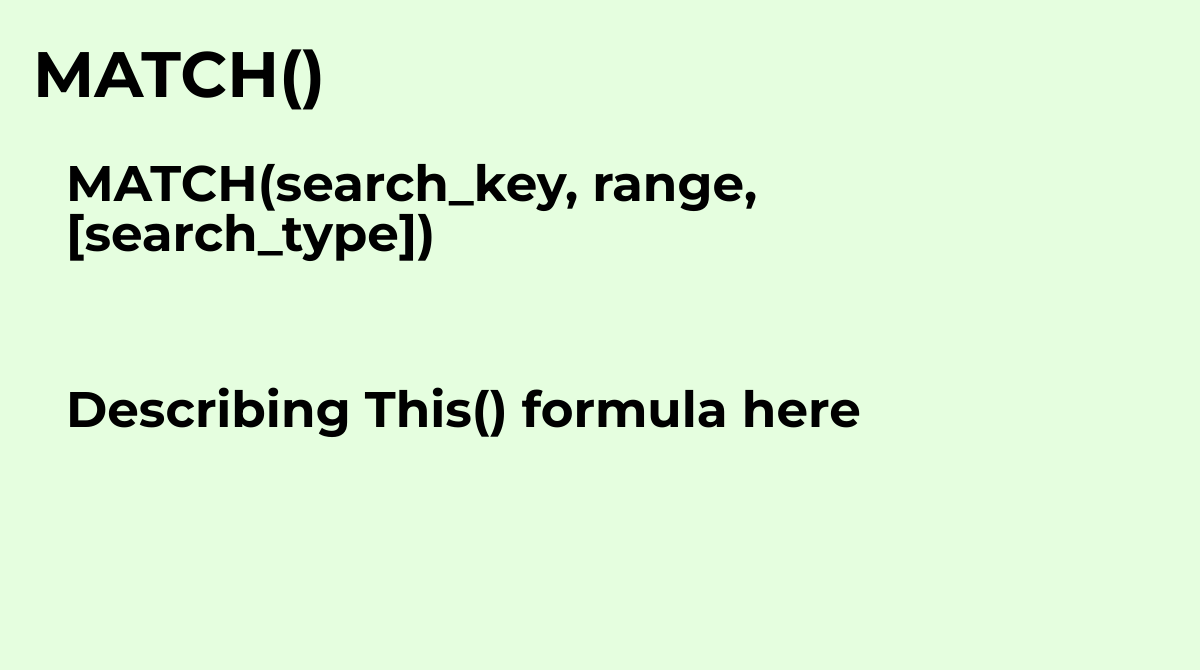Returns the relative position of an item in a range that matches a specified value.Common questio...
`MATCH(search_key, range, [search_type])`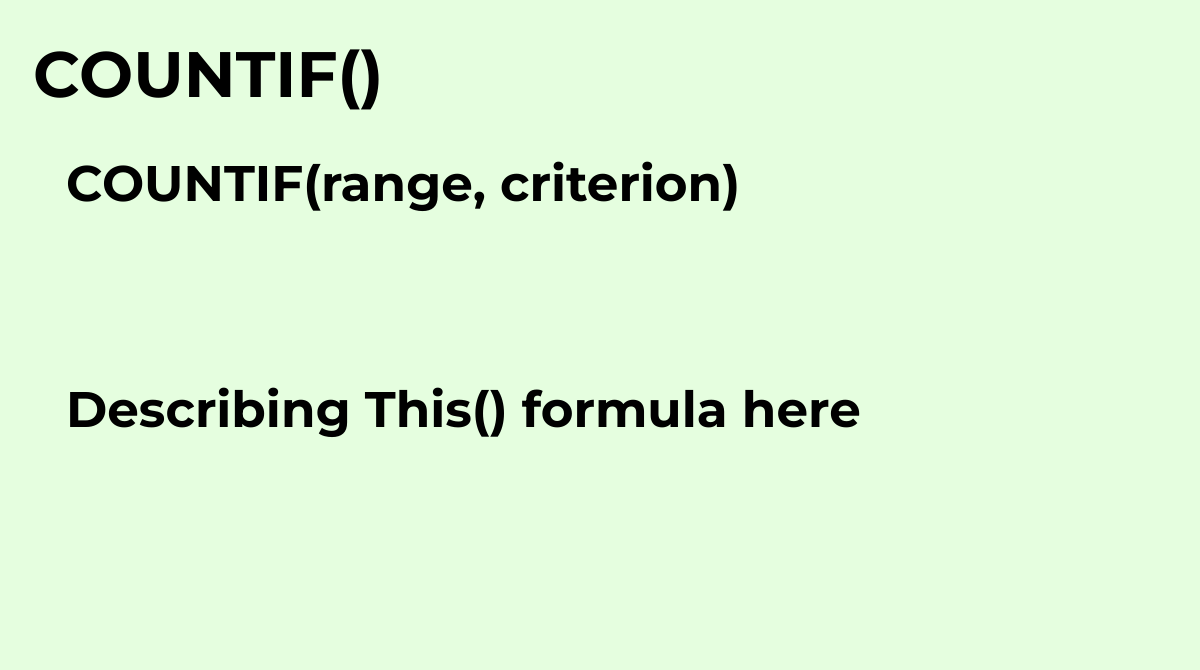Returns a conditional count across a range.Common questions about the COUNTIF formula: - How do y...
`COUNTIF(range, criterion)`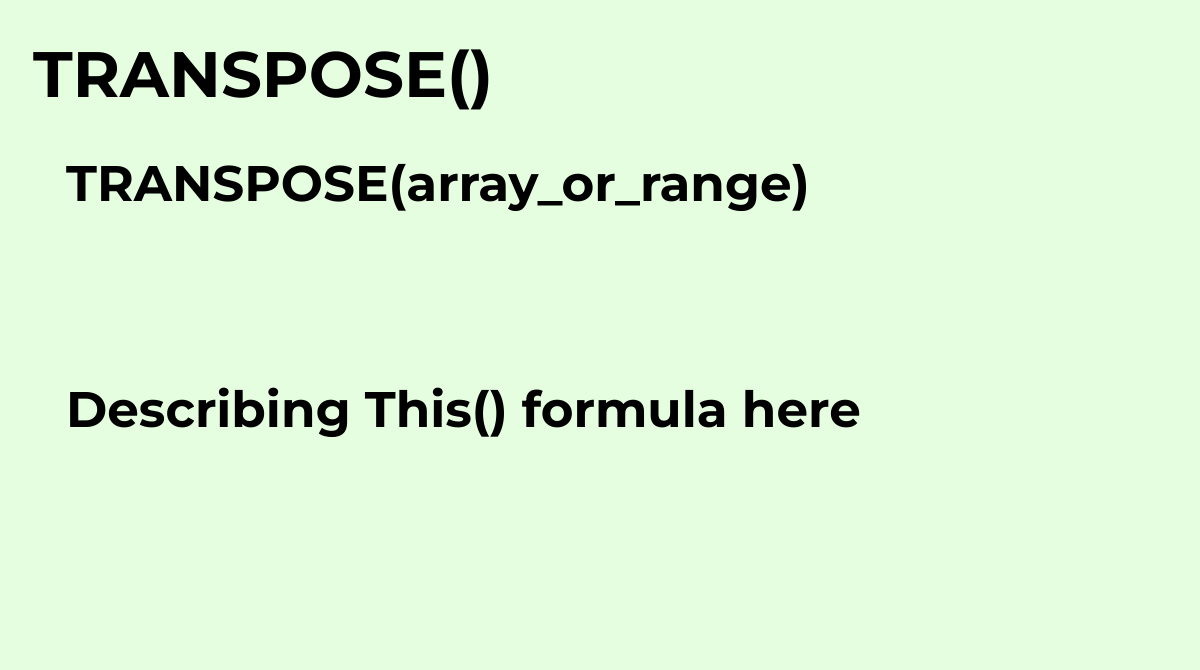Transposes the rows and columns of an array or range of cells.Common questions about the TRANSPOS...
`TRANSPOSE(array_or_range)`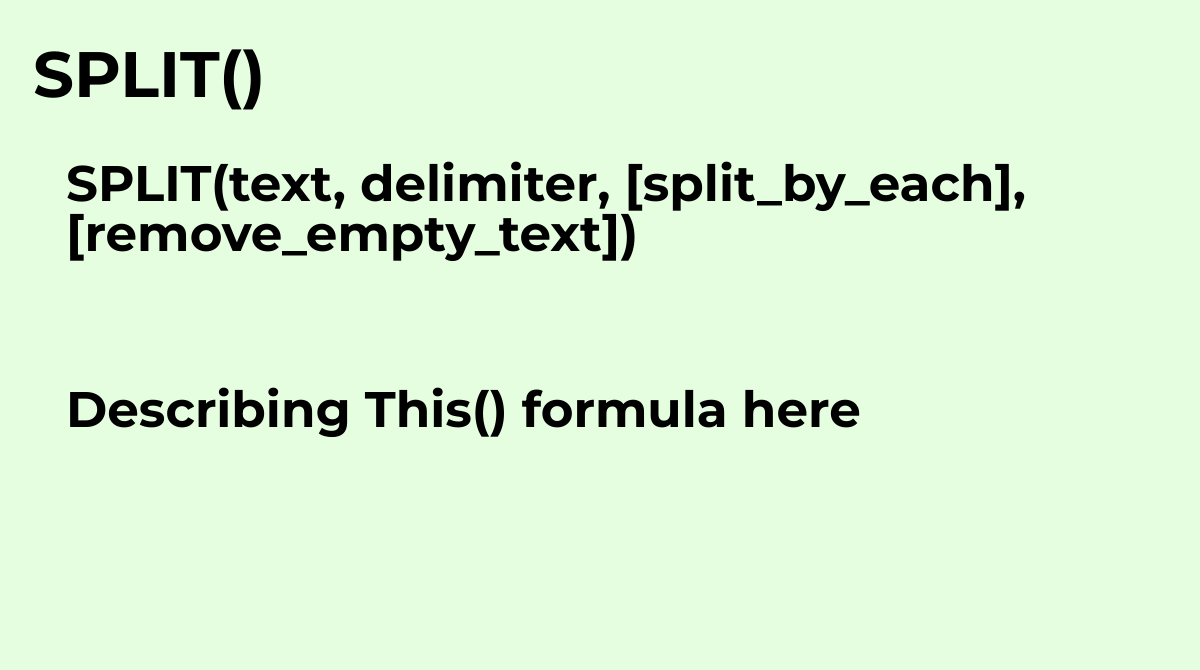Divides text around a specified character or string, and puts each fragment into a separate cell ...
`SPLIT(text, delimiter, [split_by_each], [remove_empty_text])`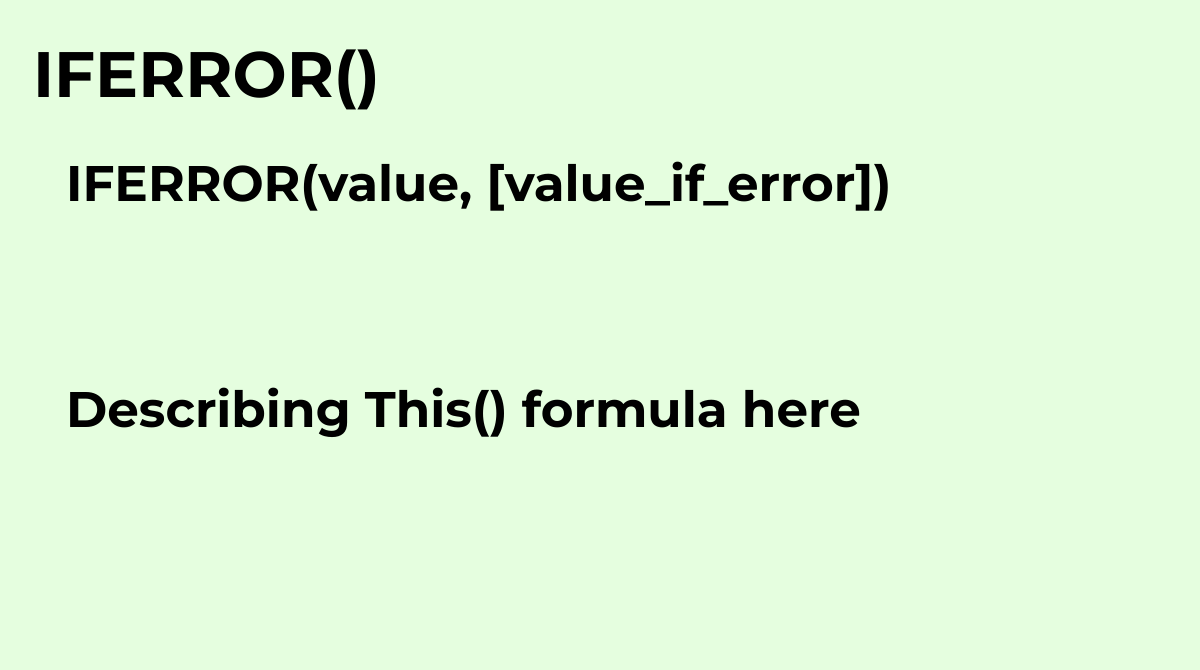Returns the first argument if it is not an error value, otherwise returns the second argument if ...
`IFERROR(value, [value_if_error])`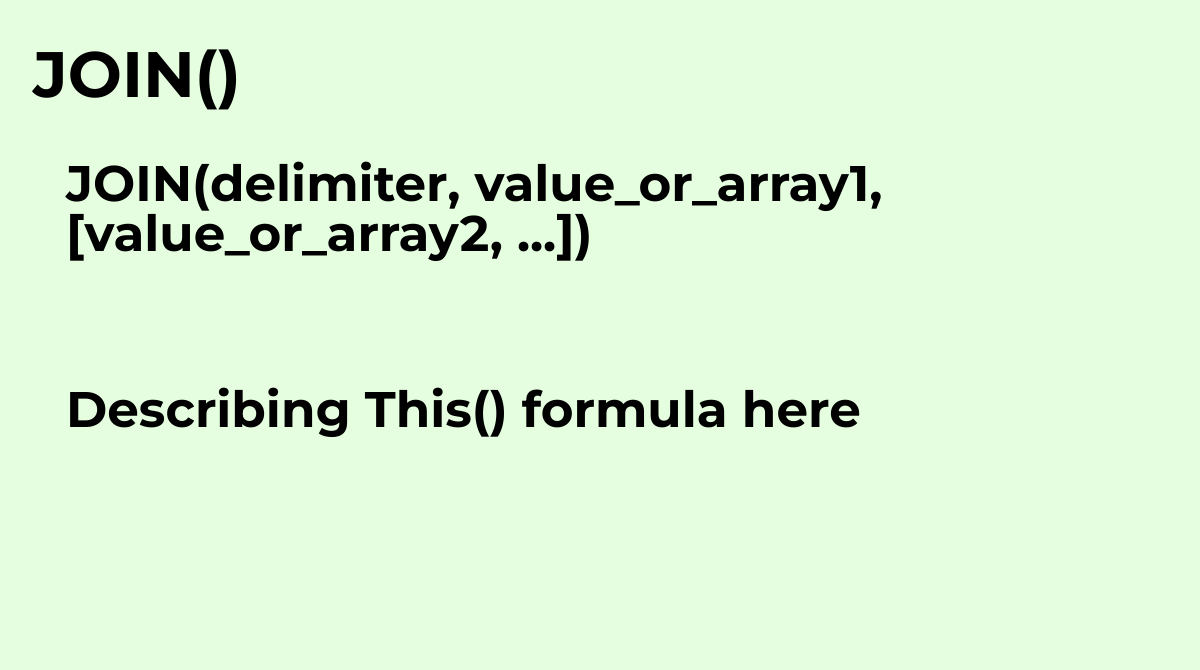Concatenates the elements of one or more one-dimensional arrays using a specified delimiter.Commo...
`JOIN(delimiter, value_or_array1, [value_or_array2, ...])`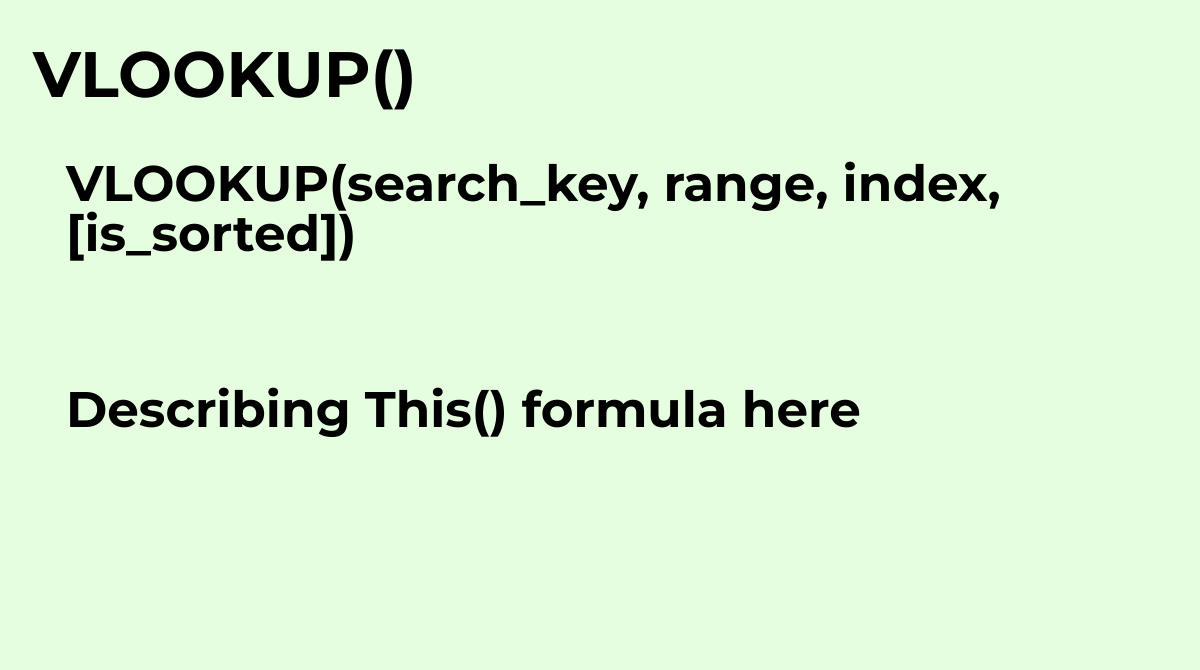Vertical lookup. Searches down the first column of a range for a key and returns the value of a s...
`VLOOKUP(search_key, range, index, [is_sorted])`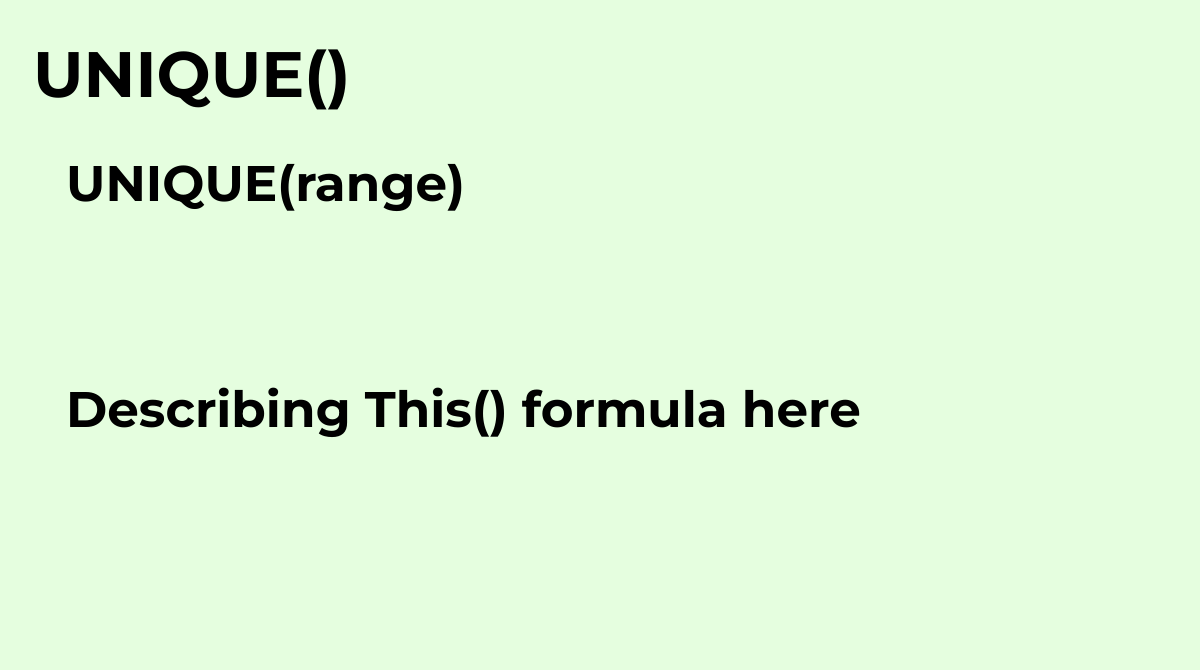Returns unique rows in the provided source range, discarding duplicates. Rows are returned in the...
`UNIQUE(range)`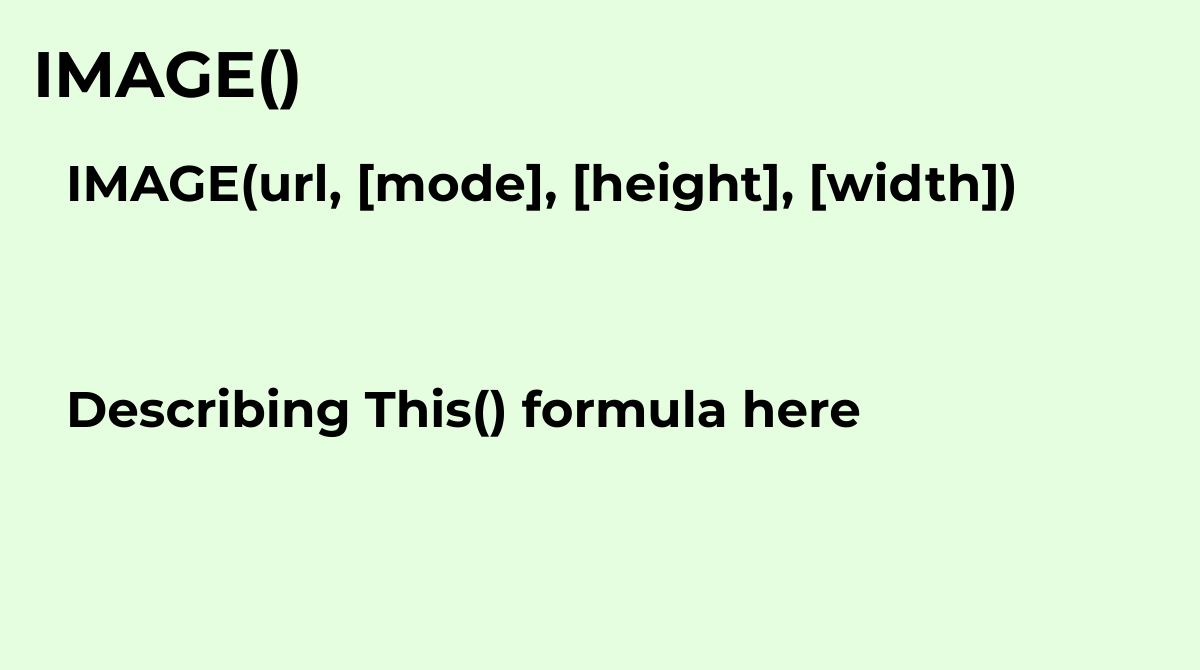Inserts an image into a cell.The IMAGE formula in Google Sheets allows you to insert an image int...
`IMAGE(url, [mode], [height], [width])`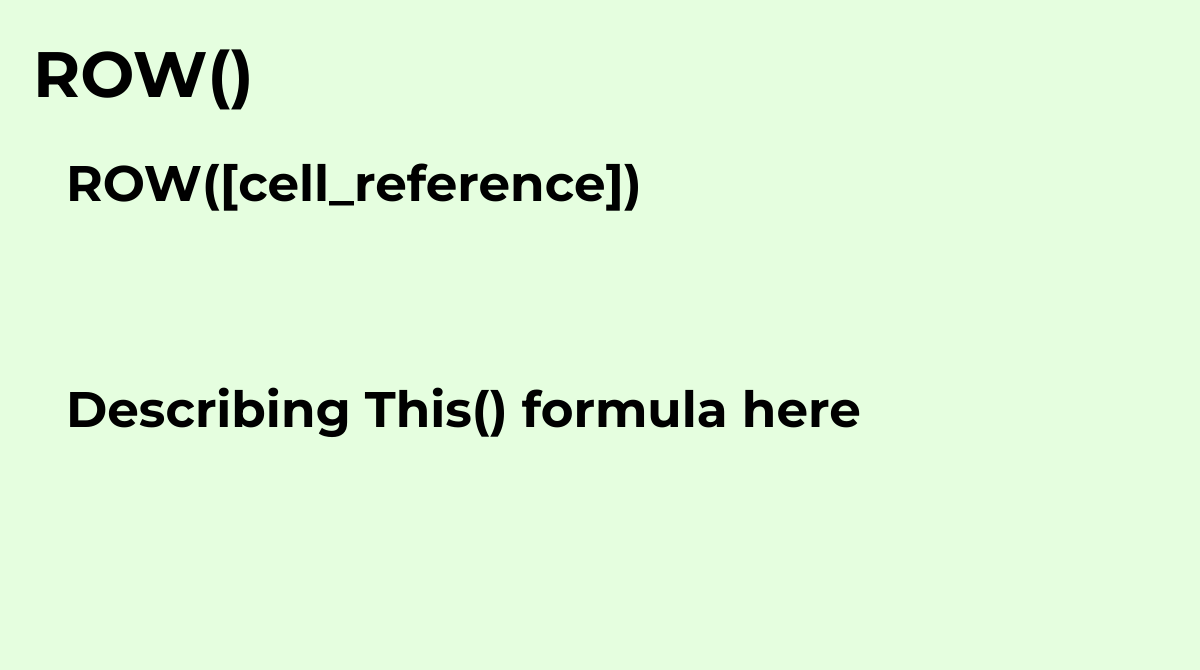Returns the row number of a specified cell.Common questions about the ROW formula in Google Sheet...
`ROW([cell_reference])`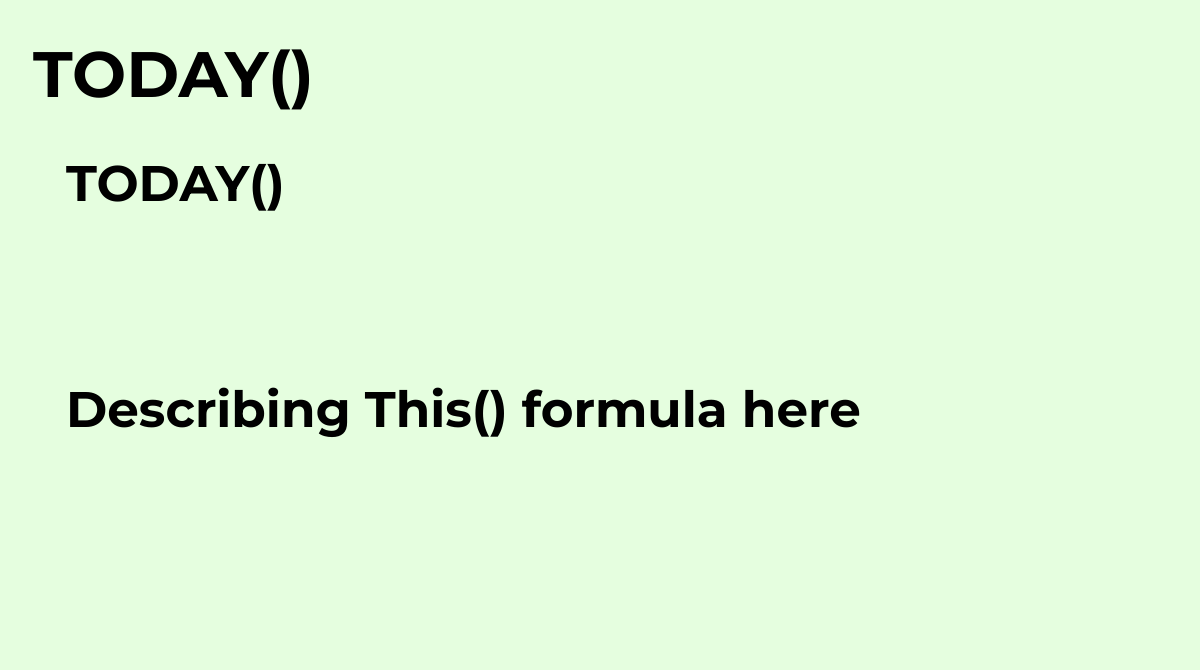Returns the current date as a date value.Common questions about the TODAY formula include:• What ...
`TODAY()`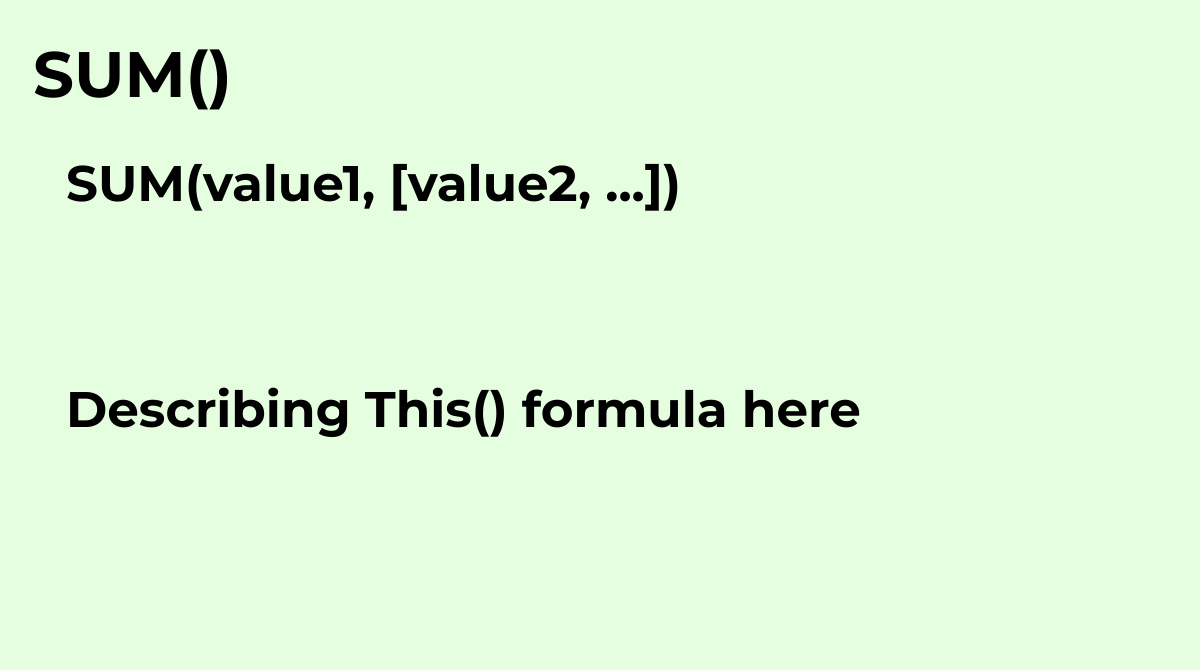Returns the sum of a series of numbers and/or cells.Common questions about the SUM formula includ...
`SUM(value1, [value2, ...])`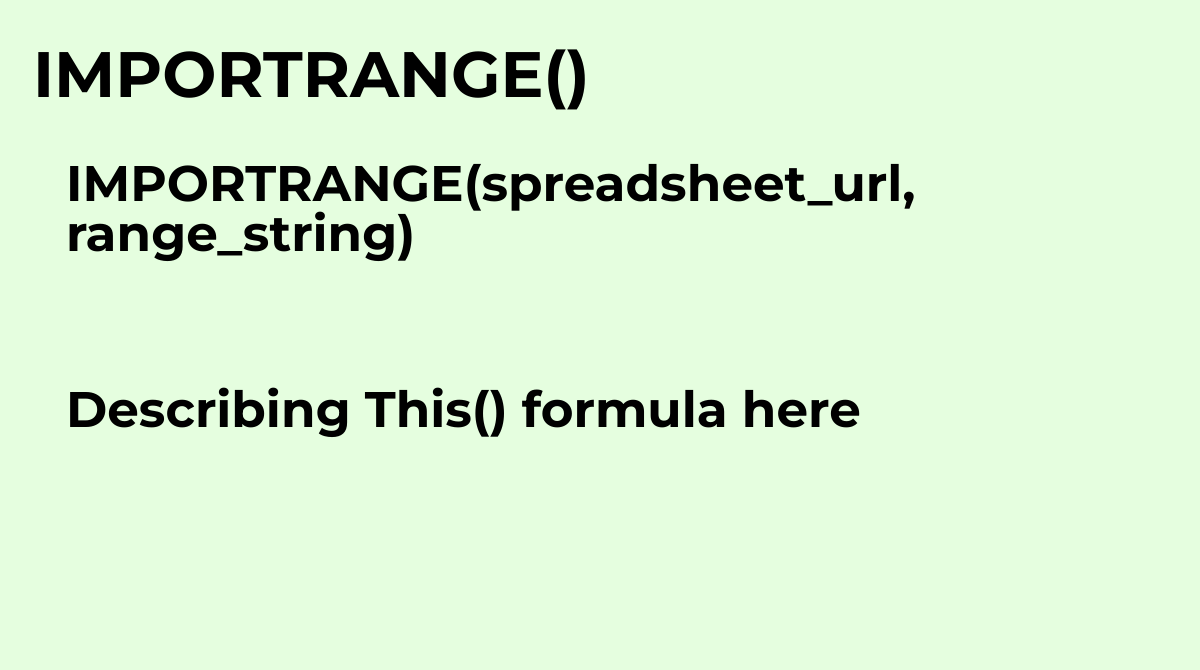Imports a range of cells from a specified spreadsheet.Common questions about the IMPORTRANGE form...
`IMPORTRANGE(spreadsheet_url, range_string)`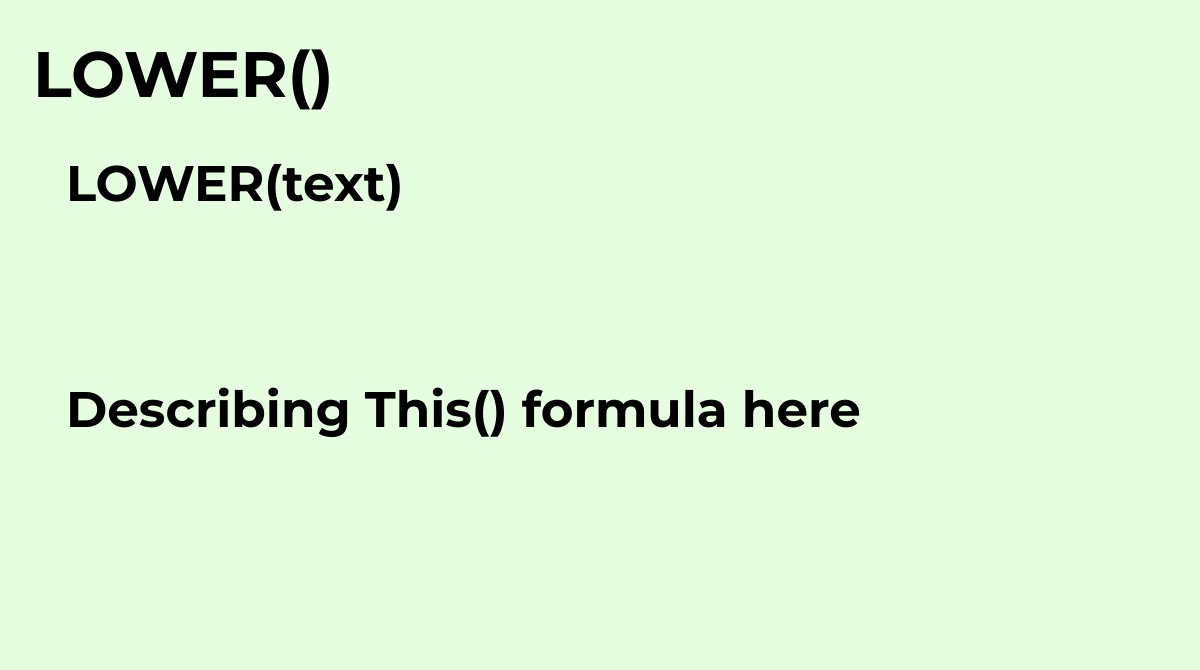Converts a specified string to lowercase.The LOWER formula in Google Sheets is a text function th...
`LOWER(text)`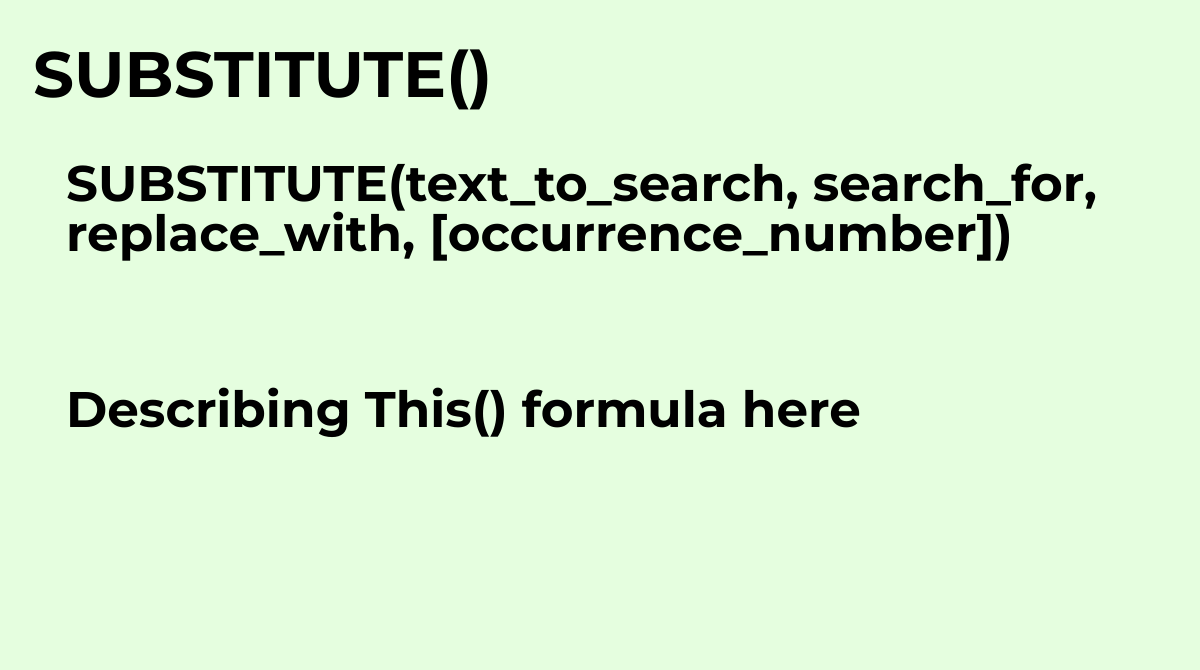Replaces existing text with new text in a string.Common questions about the SUBSTITUTE formula in...
`SUBSTITUTE(text_to_search, search_for, replace_with, [occurrence_number])`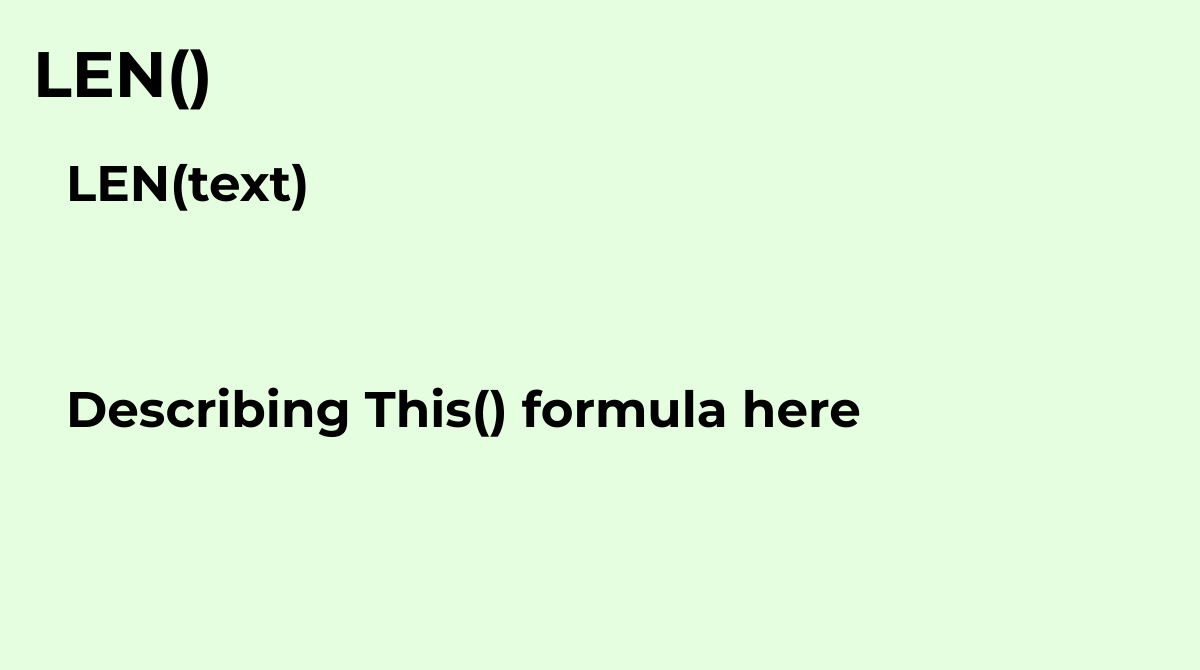Returns the length of a string.What are the common questions about the LEN formula?What does the ...
`LEN(text)`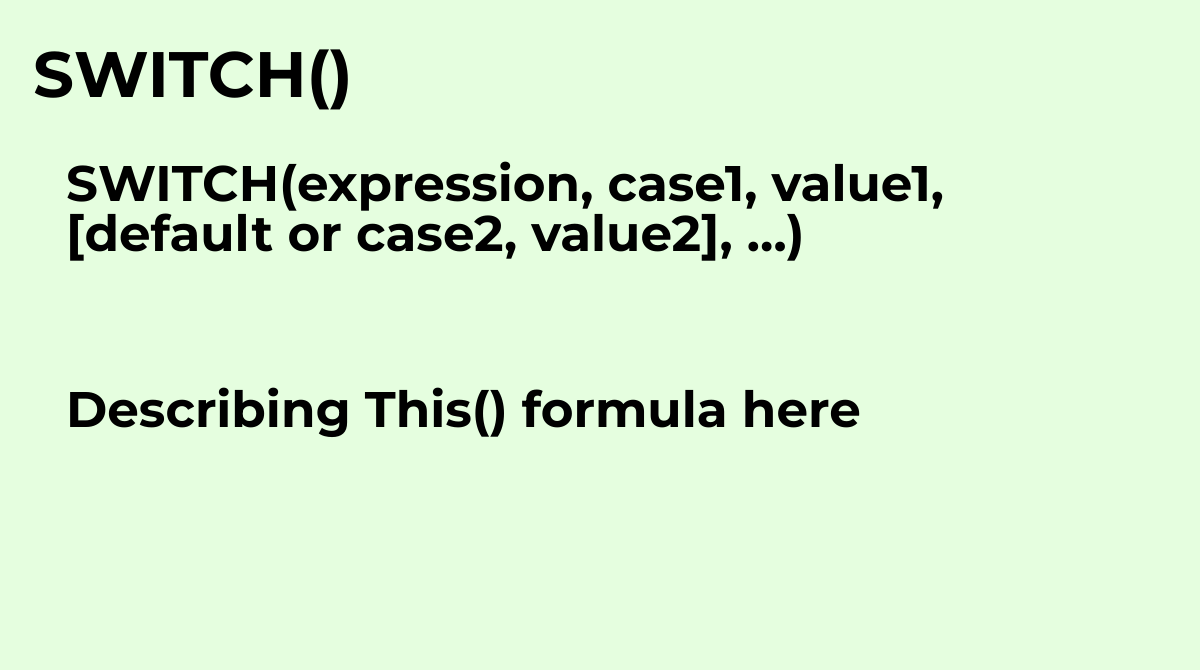Tests an expression against a list of cases and returns the corresponding value of the first matc...
`SWITCH(expression, case1, value1, [default or case2, value2], …)`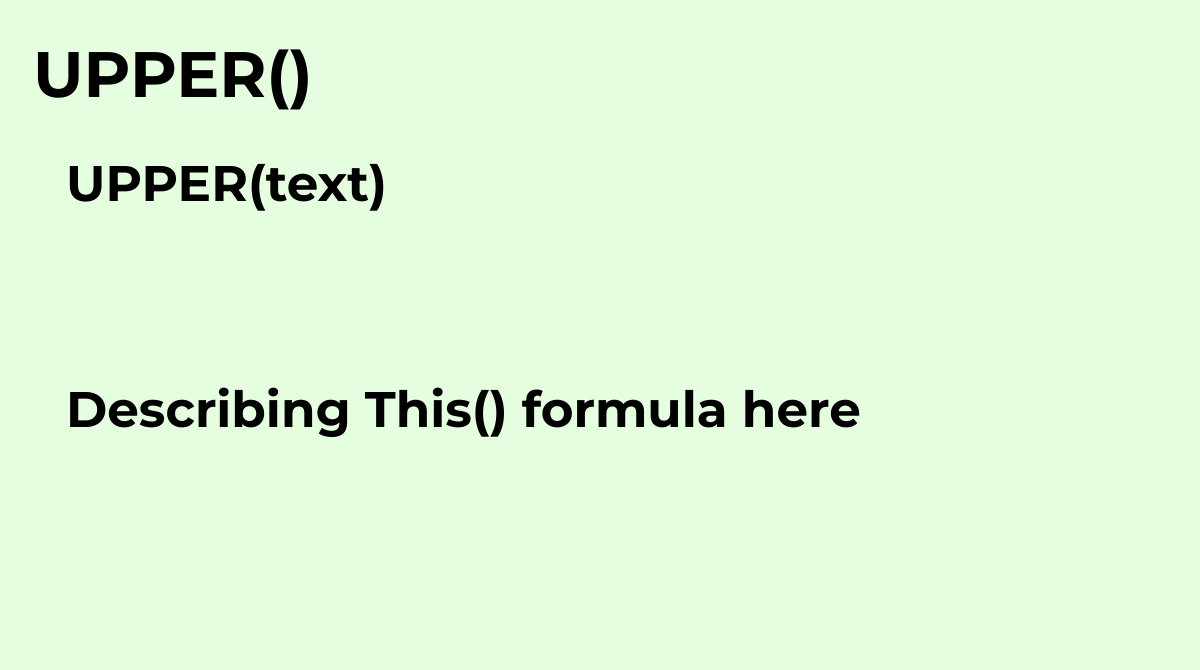Converts a specified string to uppercase.Common questions about the UPPER formula in Google Sheet...
`UPPER(text)`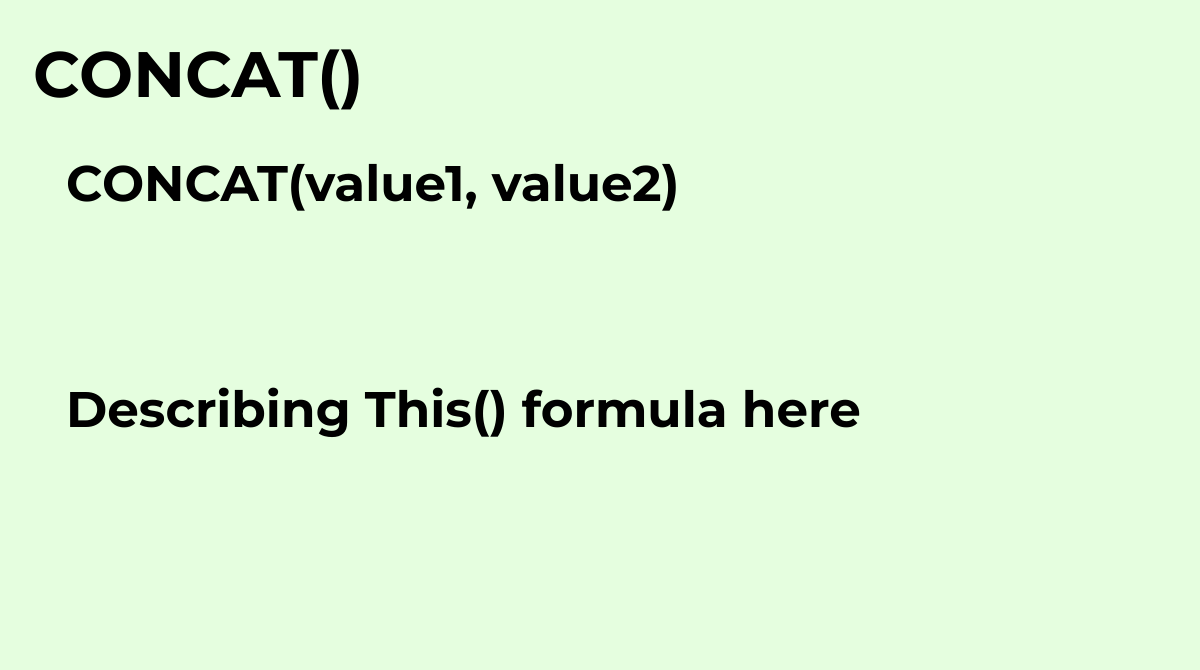Returns the concatenation of two values. The same as the `&` operator. Combines two values in...
`CONCAT(value1, value2)`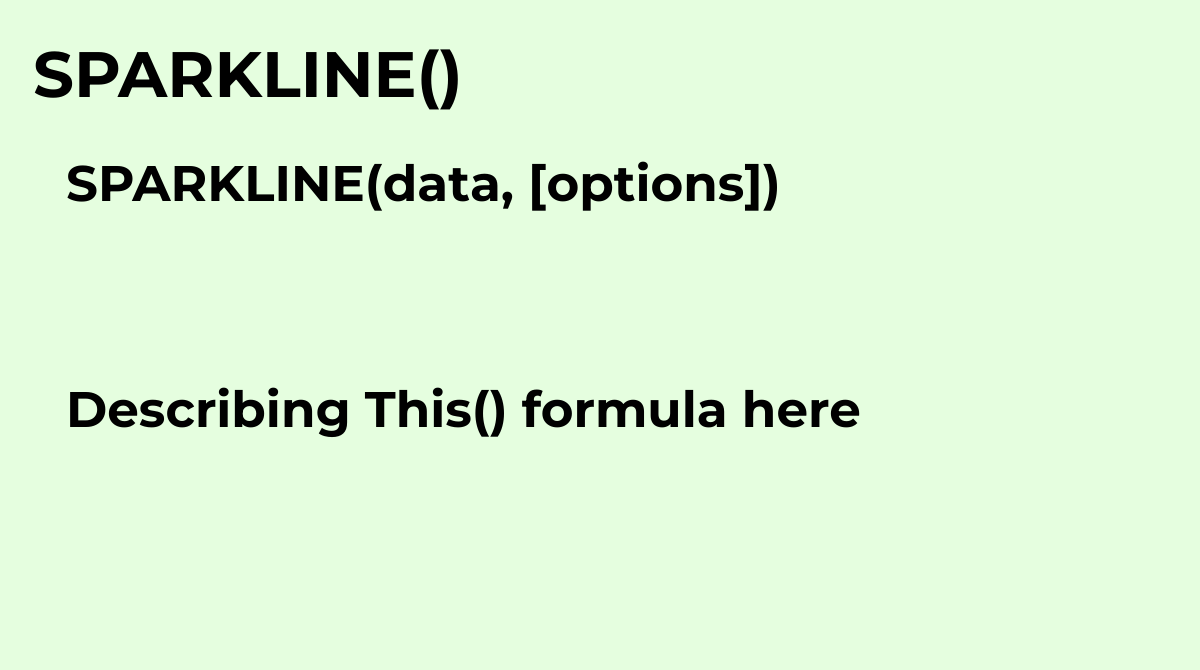Creates a miniature chart contained within a single cell.Common questions about the SPARKLINE for...
`SPARKLINE(data, [options])`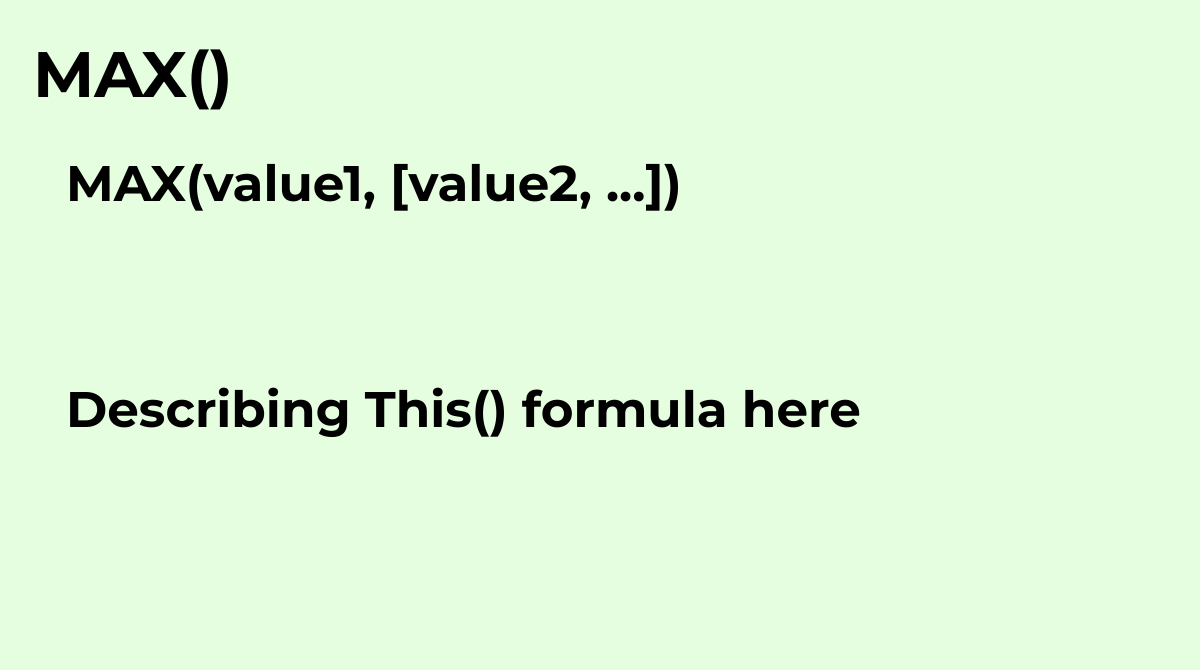Returns the maximum value in a numeric dataset.Common questions about the MAX formula in Google S...
`MAX(value1, [value2, ...])`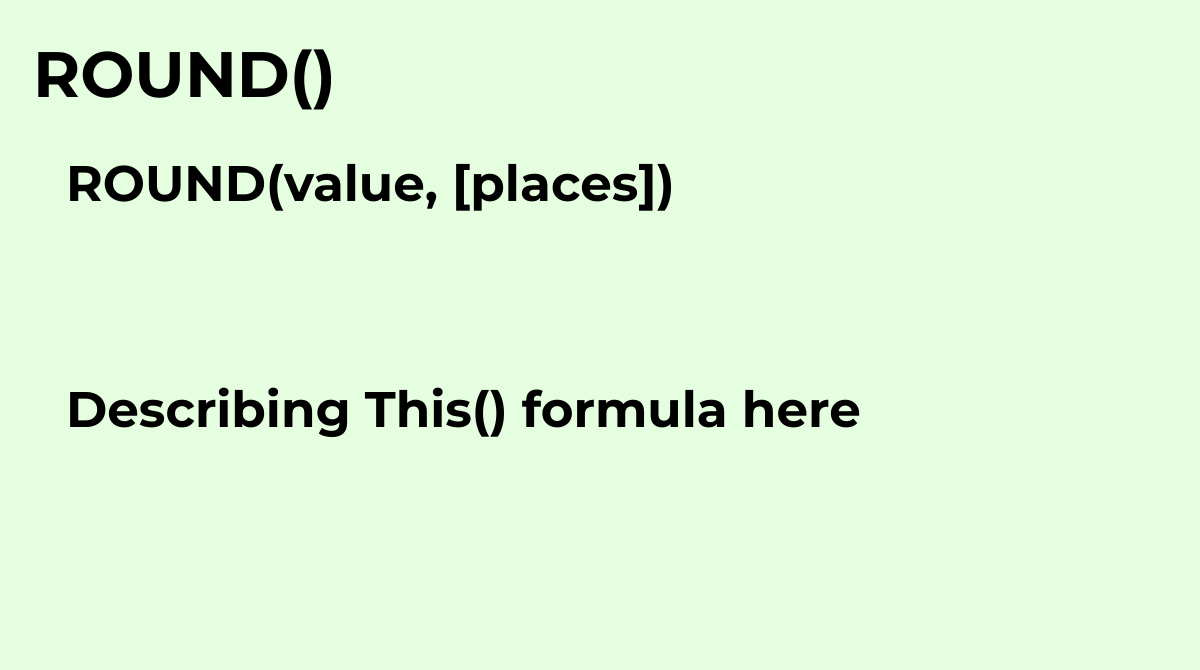Rounds a number to a certain number of decimal places according to standard rules.What are the co...
`ROUND(value, [places])`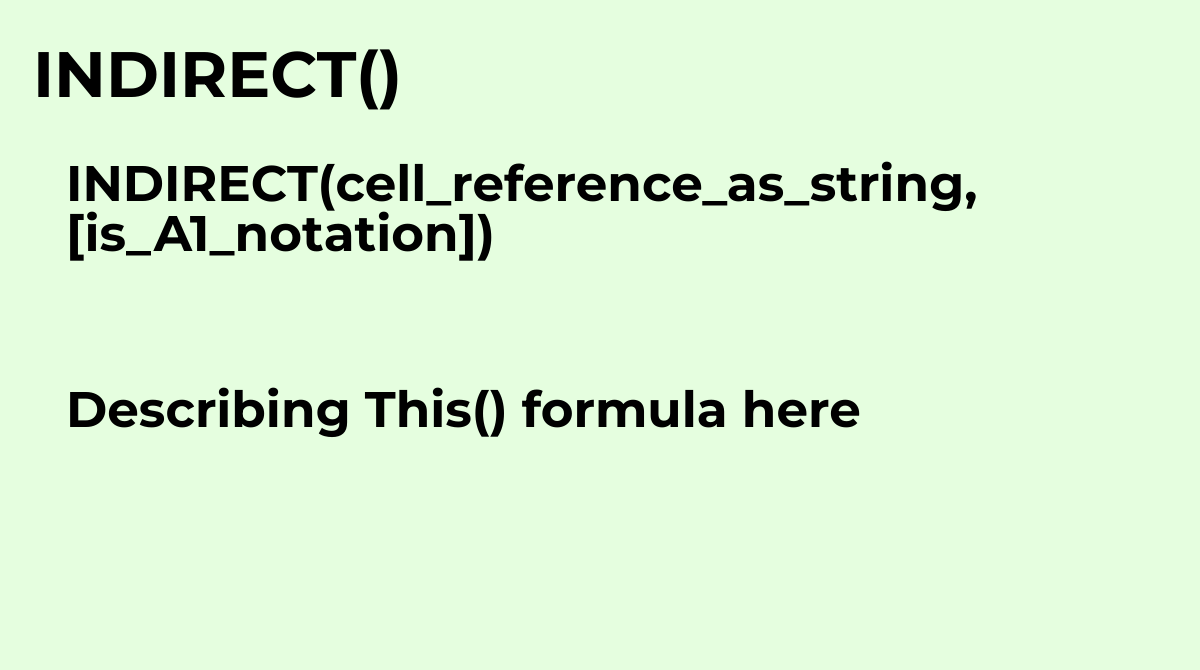Returns a cell reference specified by a string.Common questions about the INDIRECT formula: 1. Wh...
`INDIRECT(cell_reference_as_string, [is_A1_notation])`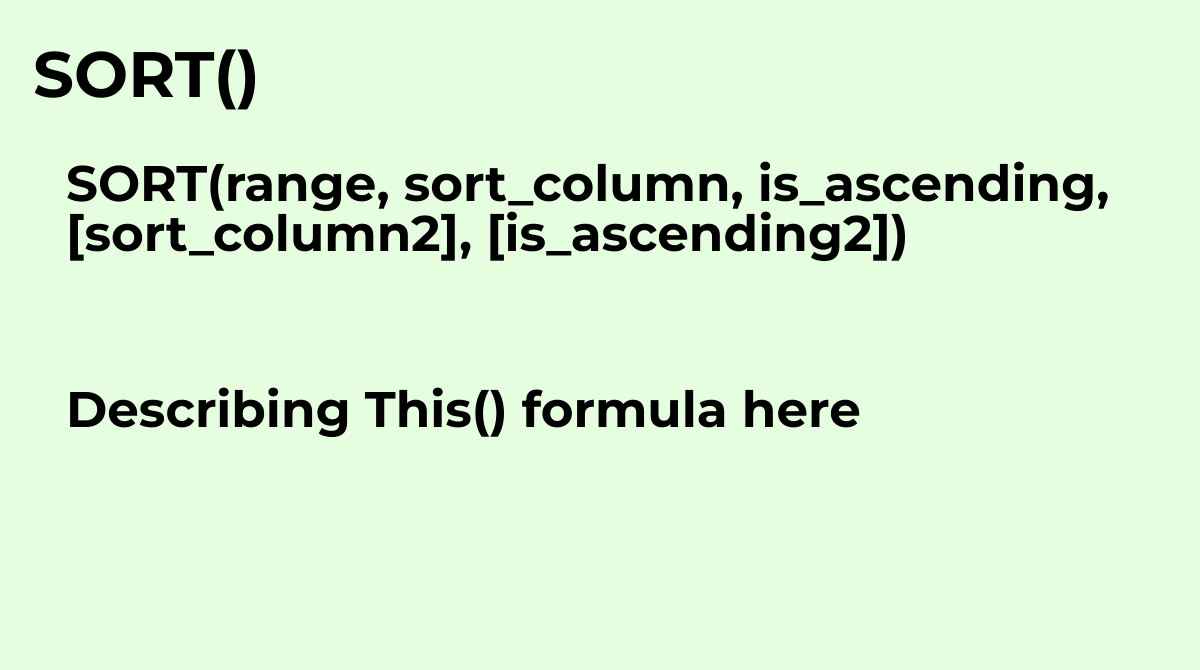Sorts the rows of a given array or range by the values in one or more columns.The SORT formula ca...
`SORT(range, sort_column, is_ascending, [sort_column2], [is_ascending2])`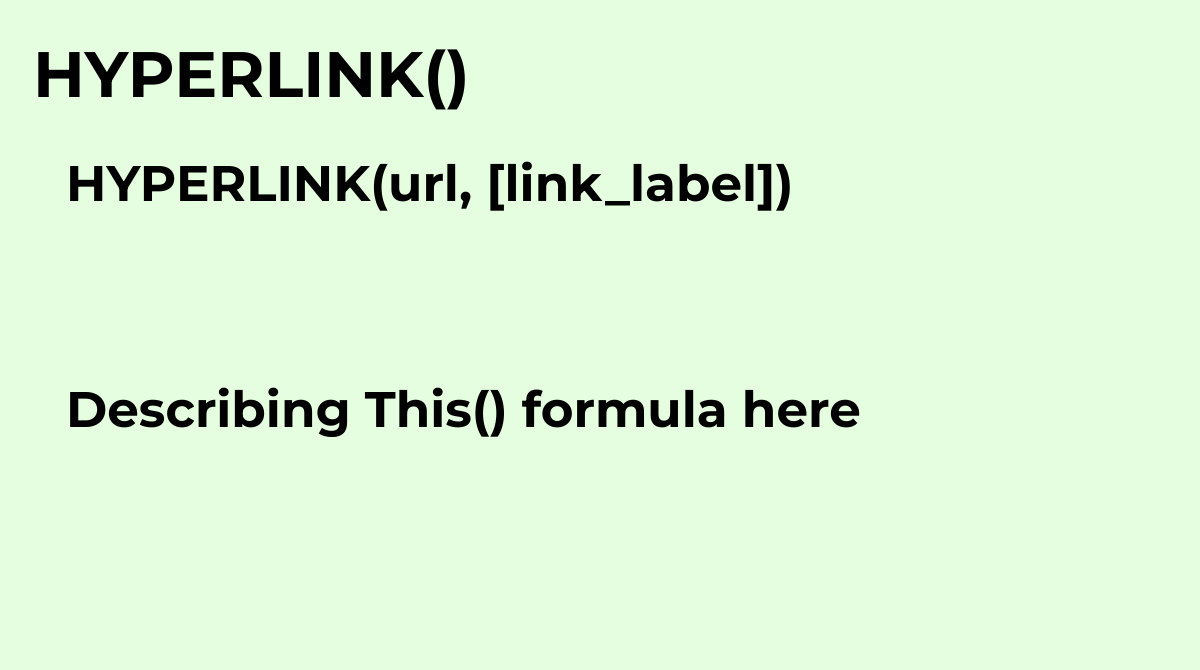`HYPERLINK(url, [link_label])`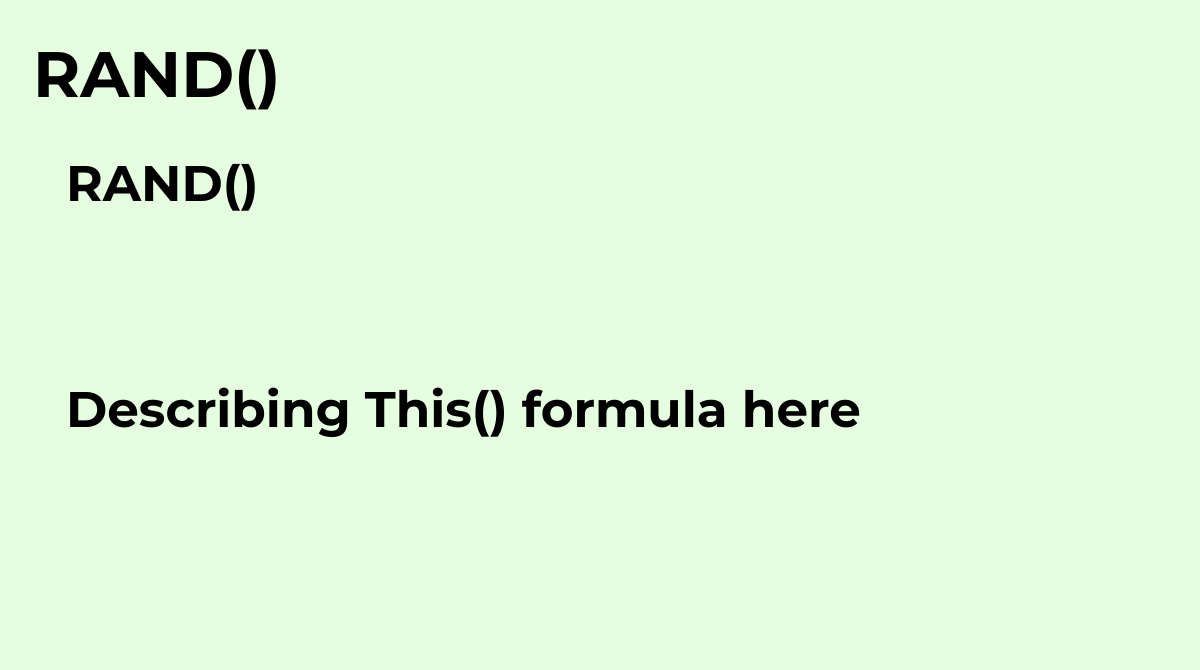Returns a random number between 0 inclusive and 1 exclusive.The RAND formula in Google Sheets is ...
`RAND()`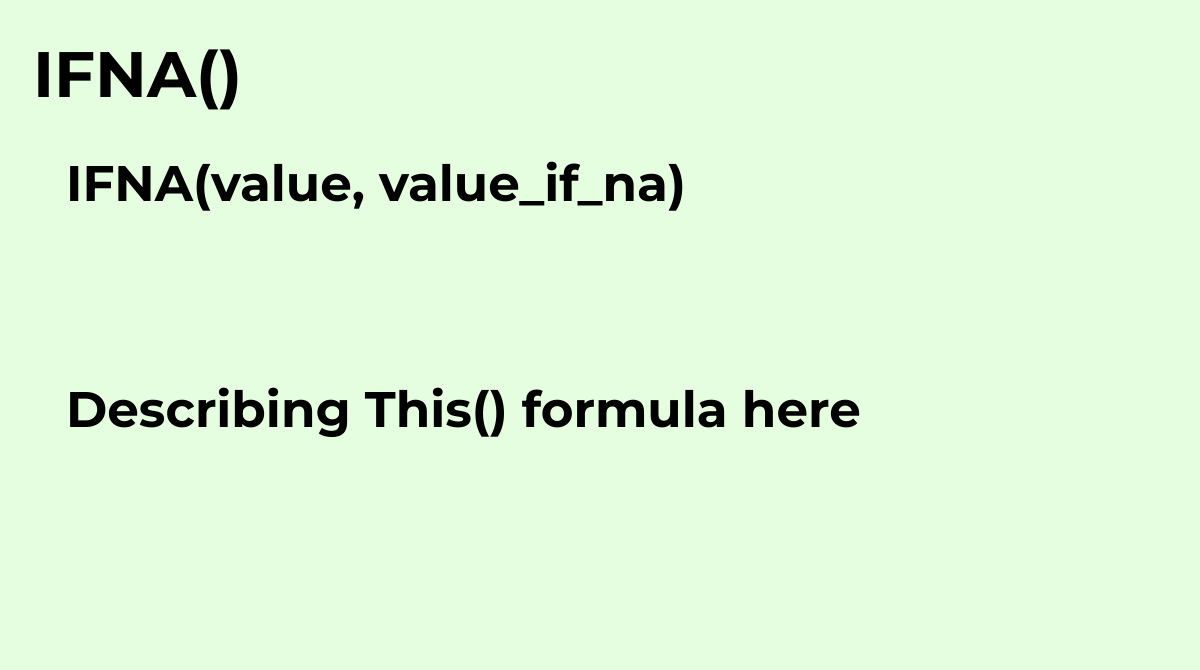Evaluates a value. If the value is an #N/A error, returns the specified value.Common questions ab...
`IFNA(value, value_if_na)`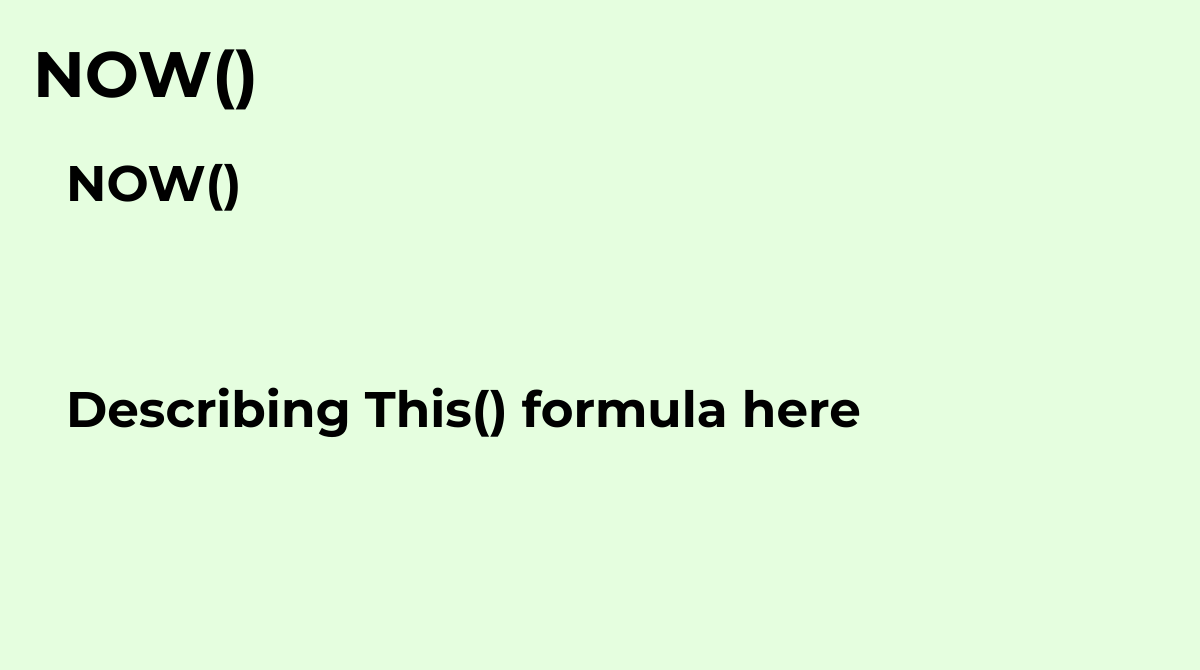Returns the current date and time as a date value.What are the common questions about the NOW for...
`NOW()`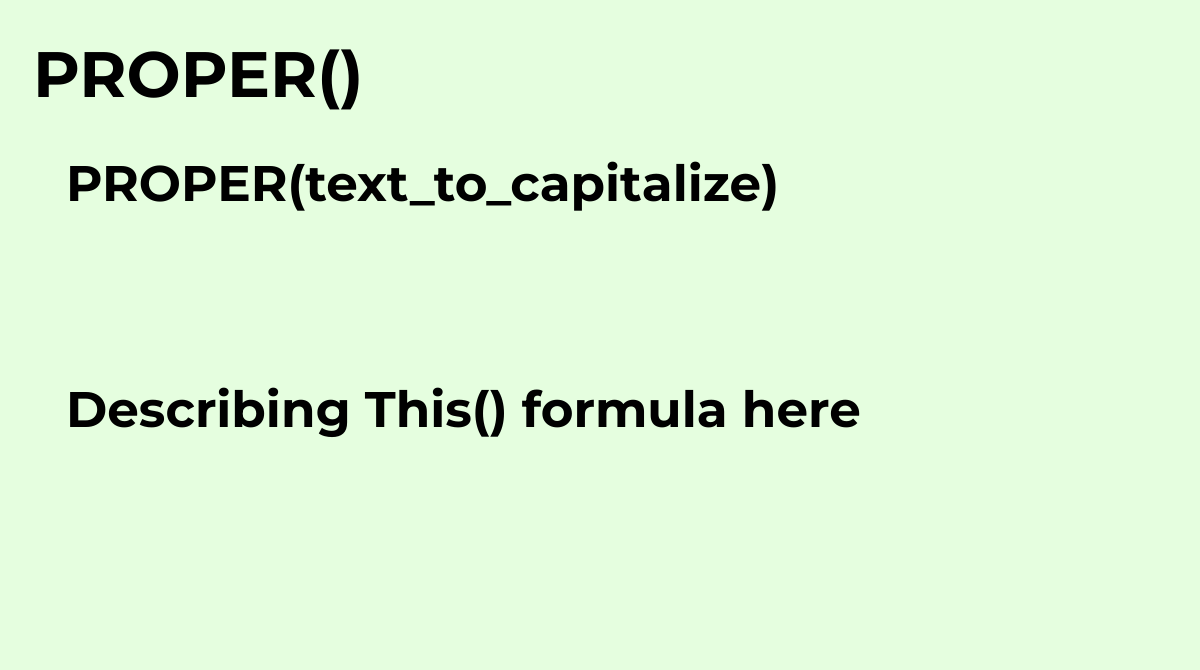Capitalizes each word in a specified string.What are the common questions about the PROPER formul...
`PROPER(text_to_capitalize)`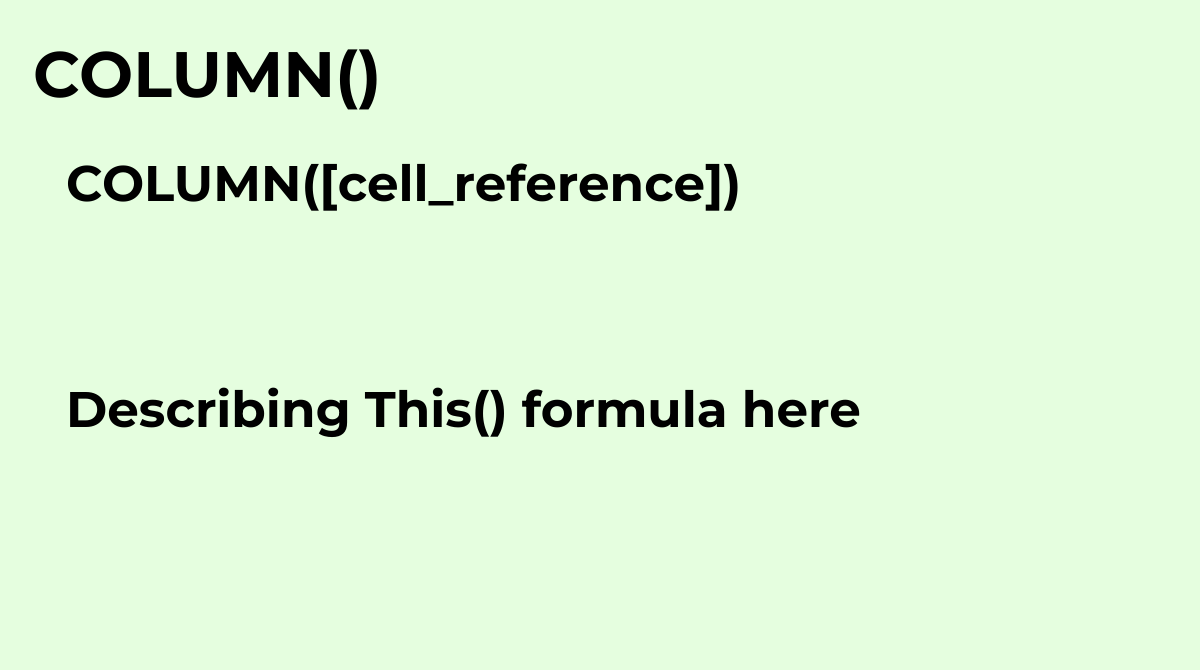Returns the column number of a specified cell, with `A=1`.What are the common questions about the...
`COLUMN([cell_reference])`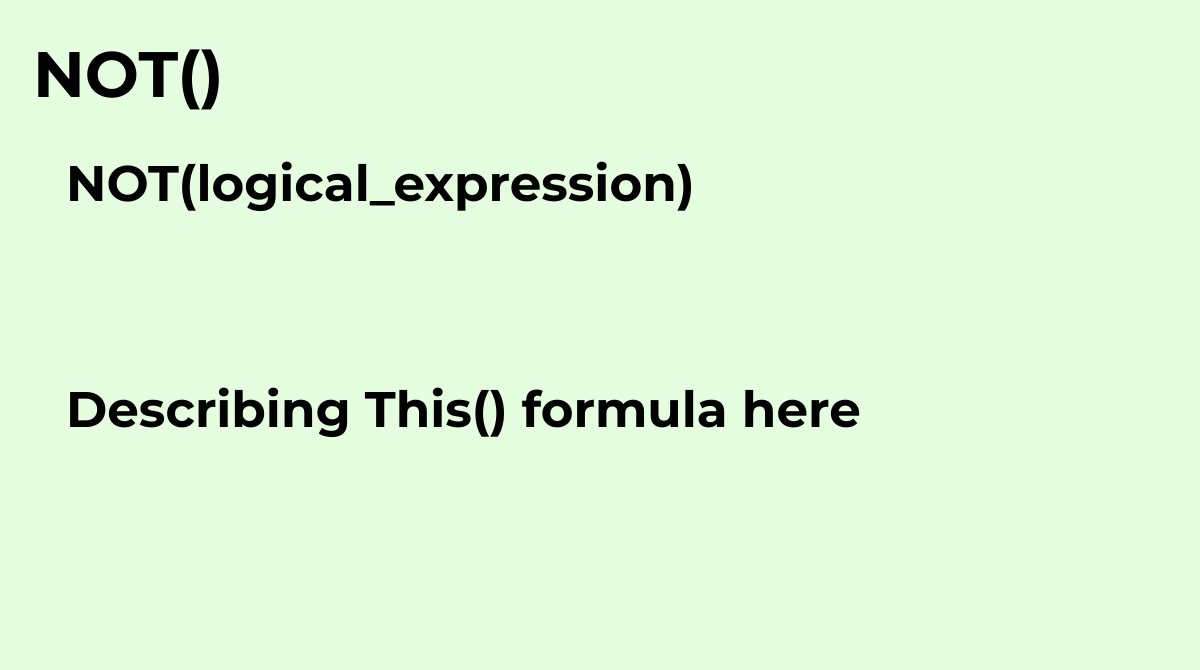Returns the opposite of a logical value - `NOT(TRUE)` returns `FALSE`; `NOT(FALSE)` returns `TRUE...
`NOT(logical_expression)`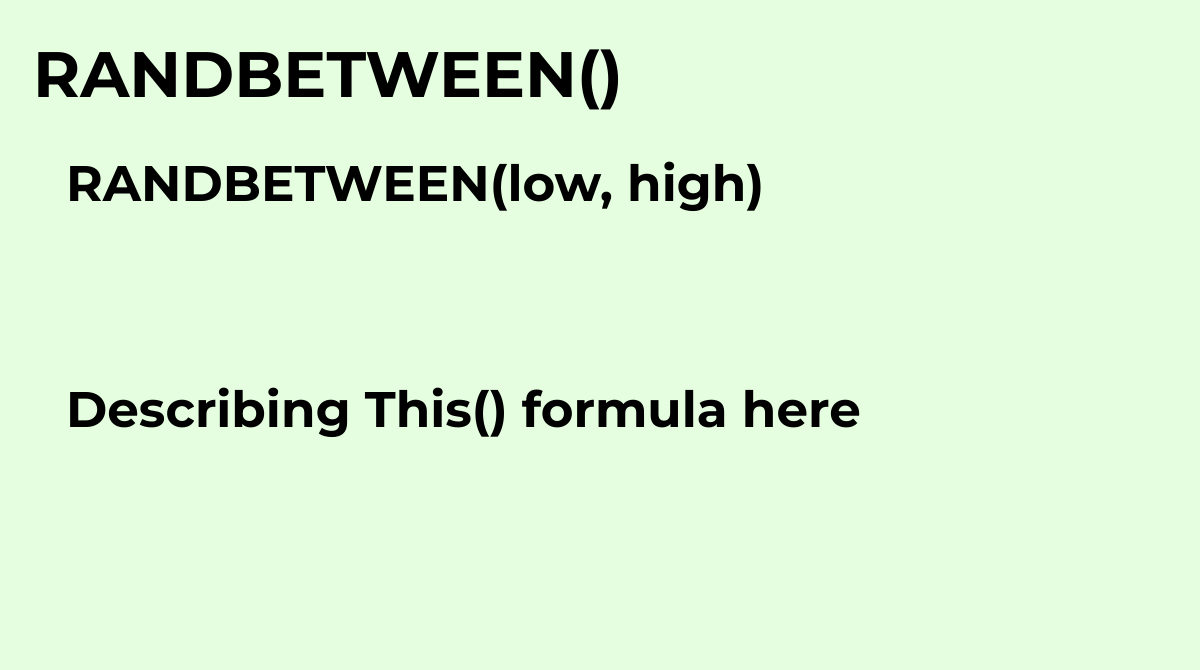Returns a uniformly random integer between two values, inclusive.Common questions about the RANDB...
`RANDBETWEEN(low, high)`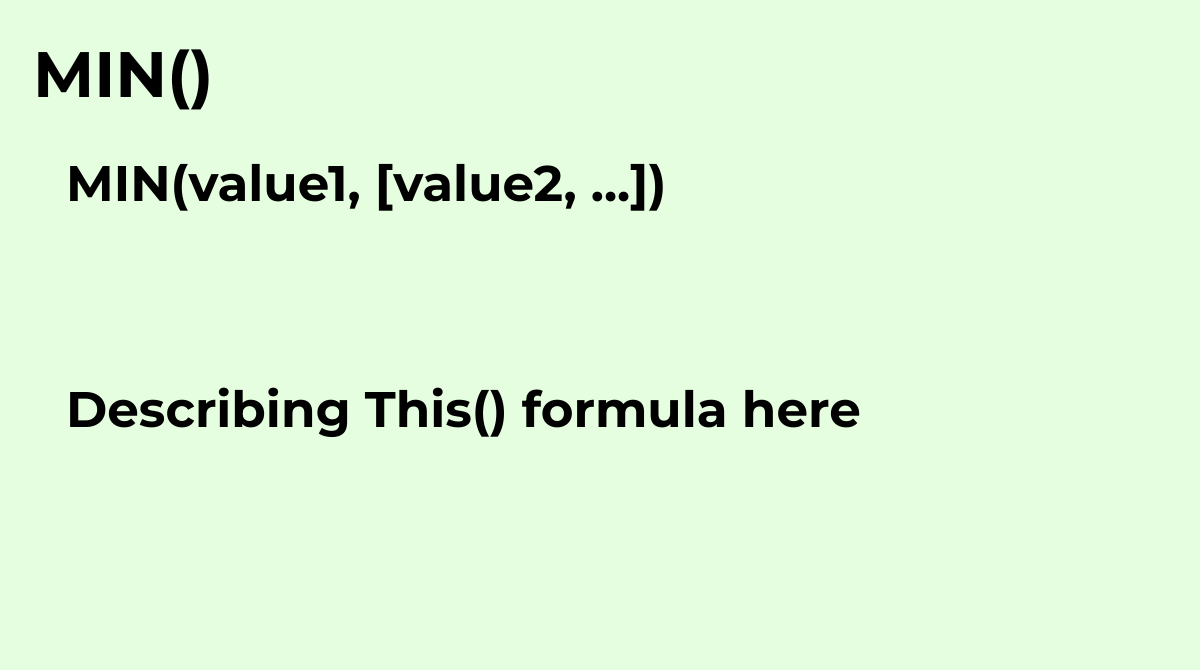Returns the minimum value in a numeric dataset.Common questions about the MIN formula in Google S...
`MIN(value1, [value2, ...])`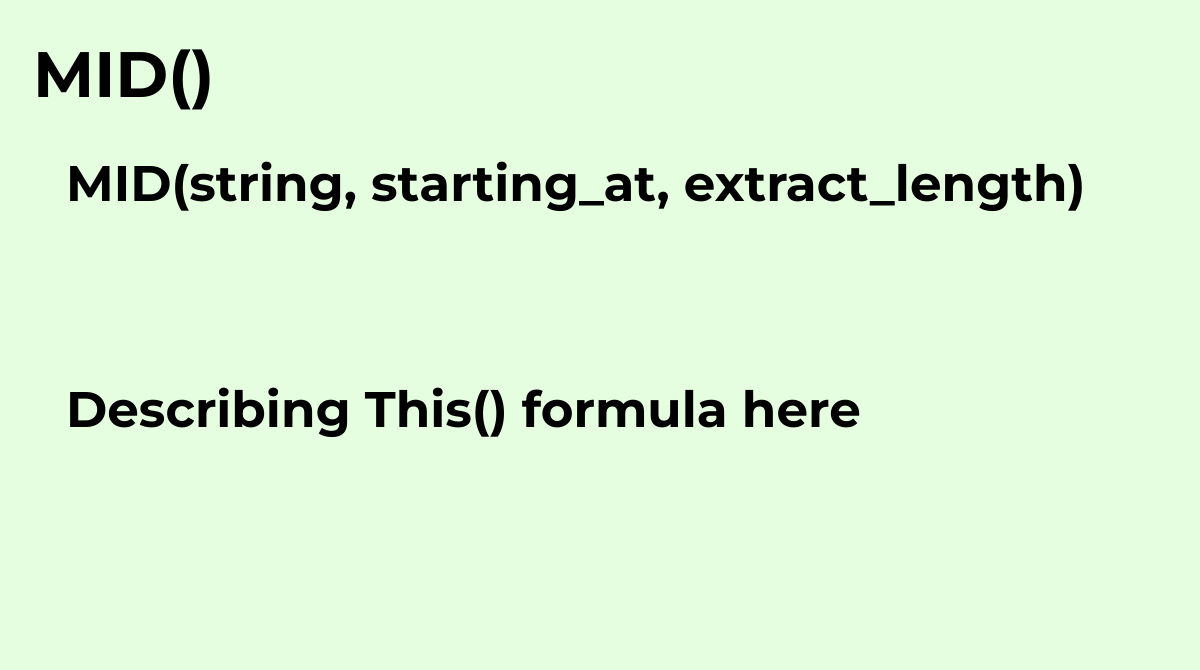Returns a segment of a string.Common questions about the MID formula in Google Sheets:What does t...
`MID(string, starting_at, extract_length)`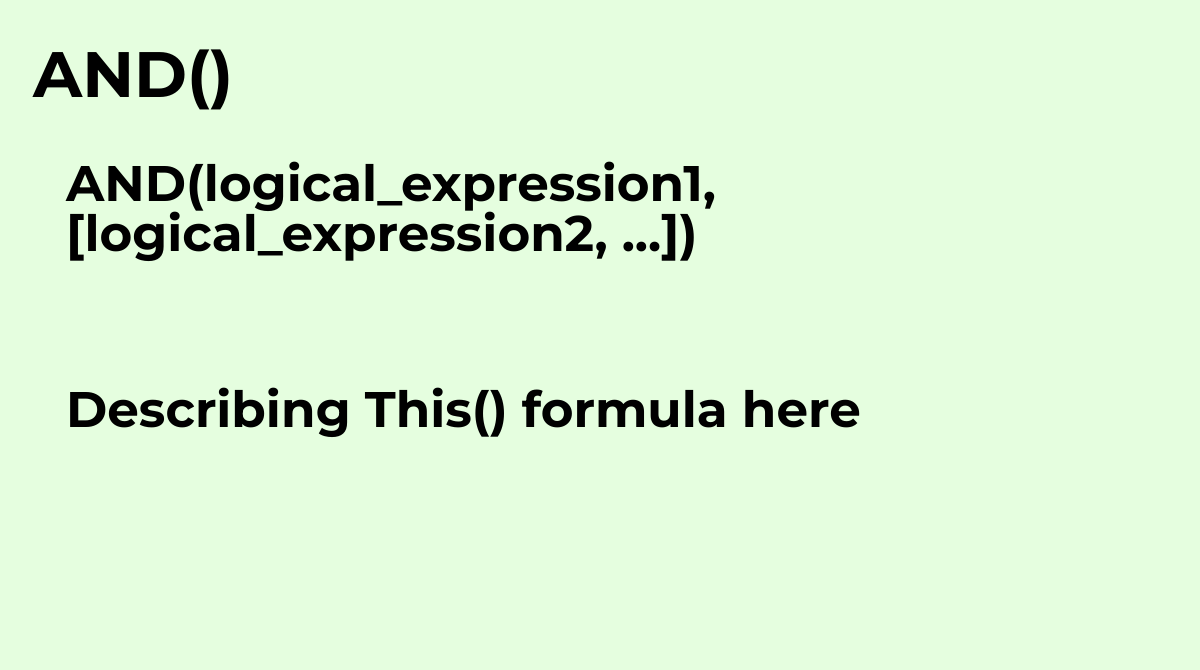Returns true if all of the provided arguments are logically true, and false if any of the provide...
`AND(logical_expression1, [logical_expression2, ...])`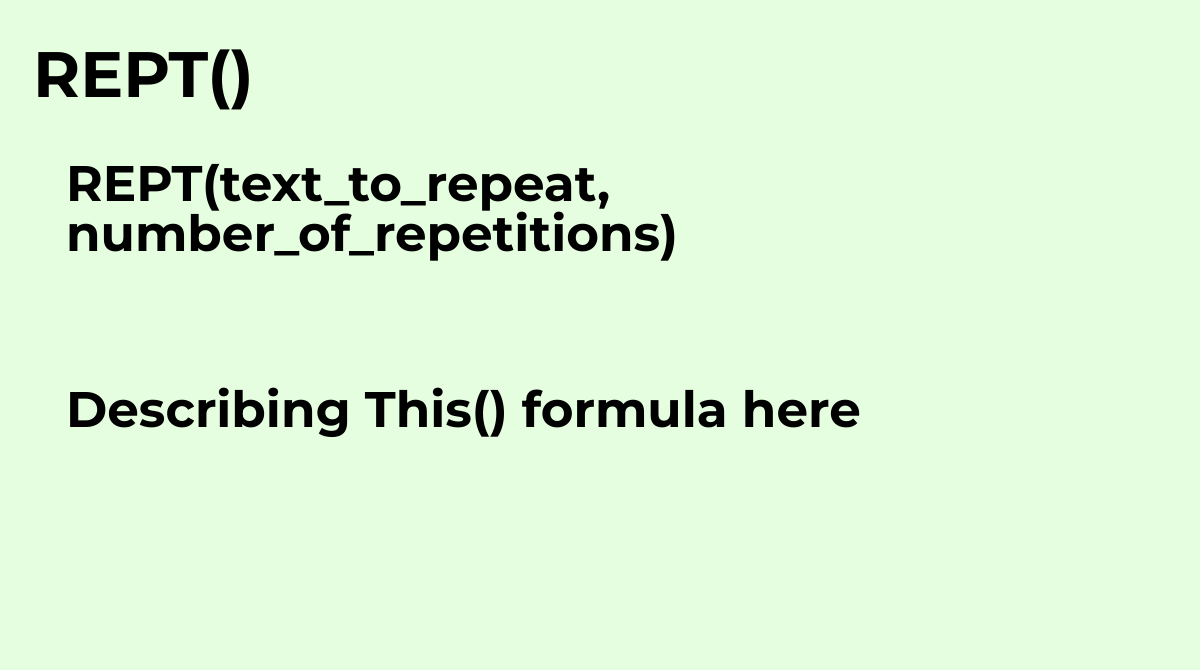Returns specified text repeated a number of times.The REPT formula in Google Sheets is used to re...
`REPT(text_to_repeat, number_of_repetitions)`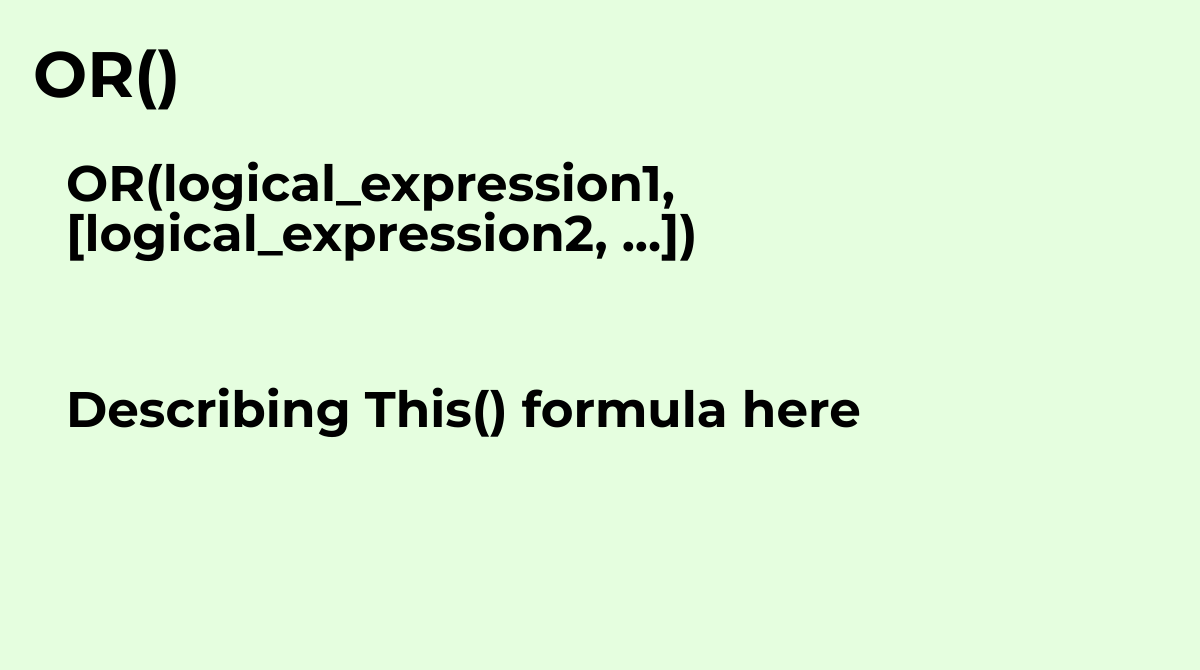Returns true if any of the provided arguments are logically true, and false if all of the provide...
`OR(logical_expression1, [logical_expression2, ...])`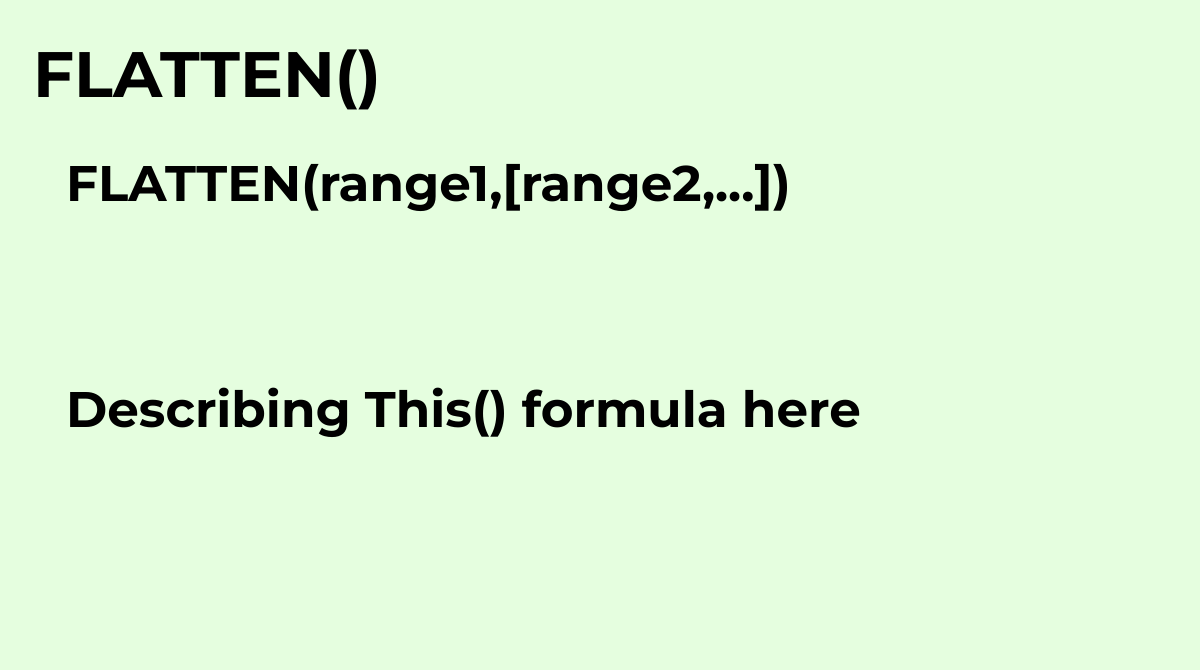Flattens all the values from one or more ranges into a single column.Common questions about the F...
`FLATTEN(range1,[range2,...])`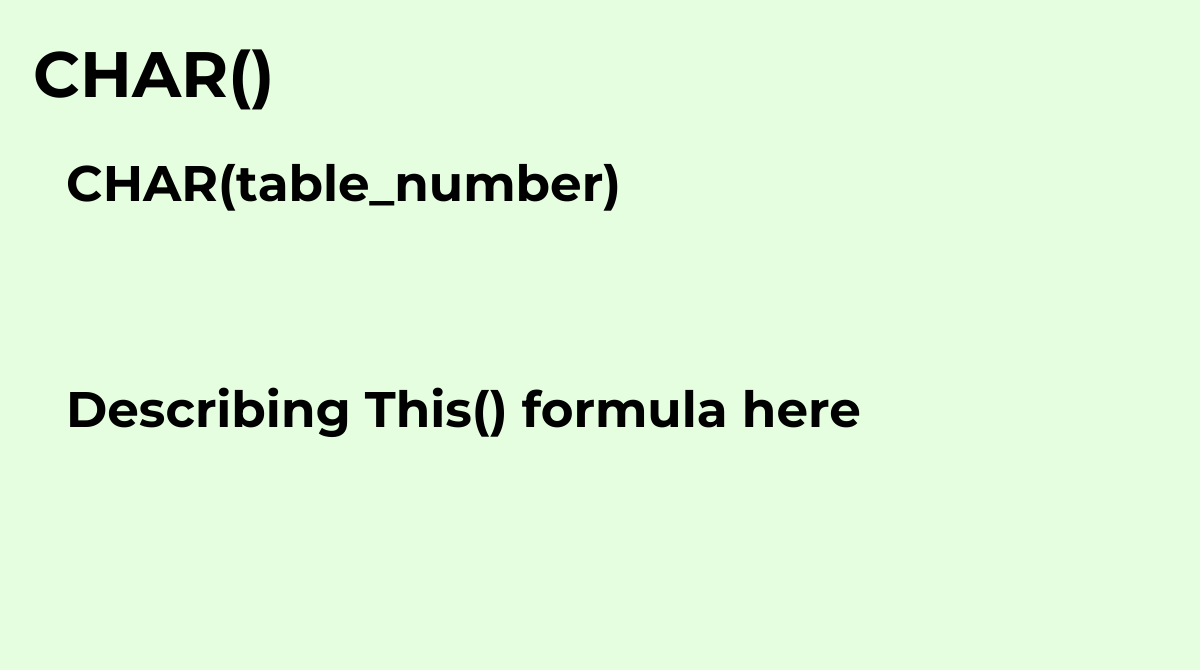Convert a number into a character according to the current Unicode table.The CHAR formula in Goog...
`CHAR(table_number)`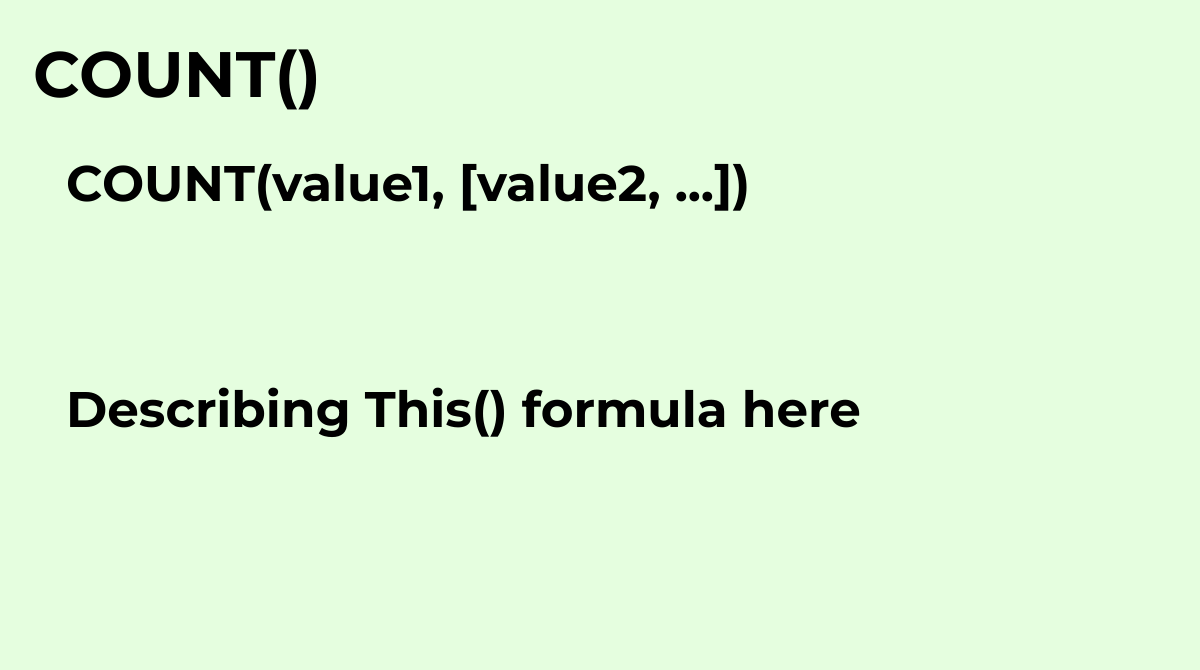Returns a count of the number of numeric values in a dataset.What are the common questions about ...
`COUNT(value1, [value2, ...])`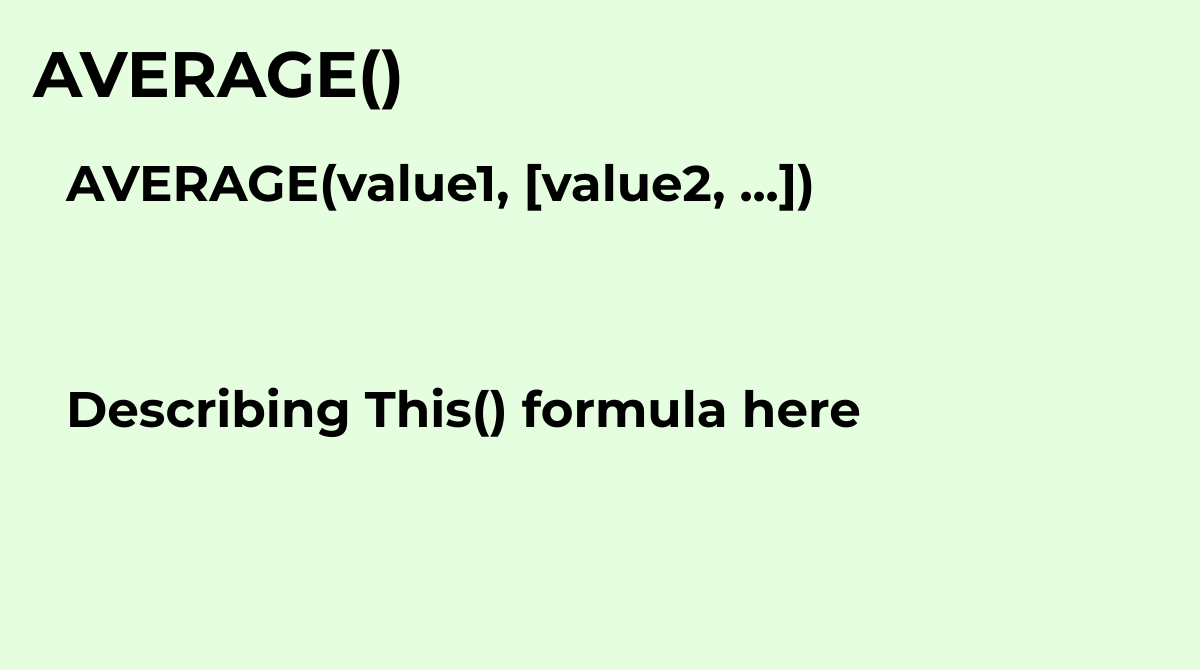Returns the numerical average value in a dataset, ignoring text.
`AVERAGE(value1, [value2, ...])`

Displaying items 1-48 of 515 in total